# MSBSHSE Solutions For SSC (Class 10) Maths Part 2 Chapter 3 - Circle

MSBSHSE Solutions For SSC (Class 10) Maths Part 2 Chapter 3 – Circle are provided here in step by step explanations. The clear diagrams given in our solutions help students better understand the concept of circle. This chapter deals with circles touching each other, secant and tangent of circle, inscribed angle, intercepted arc and arc of a circle. We also come across cyclic quadrilateral and Theorem of intersecting chords in this chapter. These MSBSHSE Solutions For SSC (Class 10) are crucial for students who are preparing for board examinations. BYJU’S provides error free solutions designed by our subject experts. These solutions can be downloaded in PDF format.

## Download the PDF of Maharashtra Solutions For SSC Maths Part 2 Chapter 3 Circle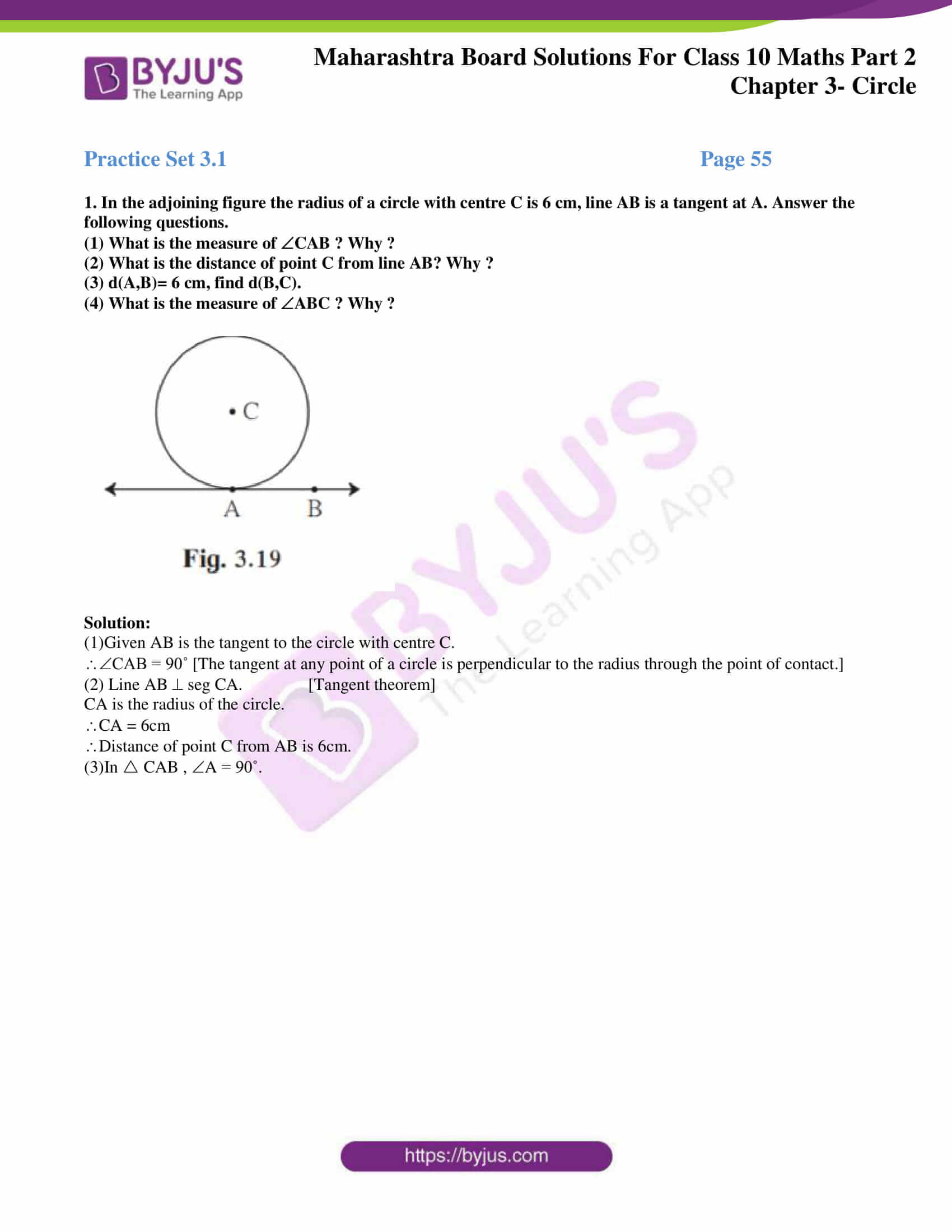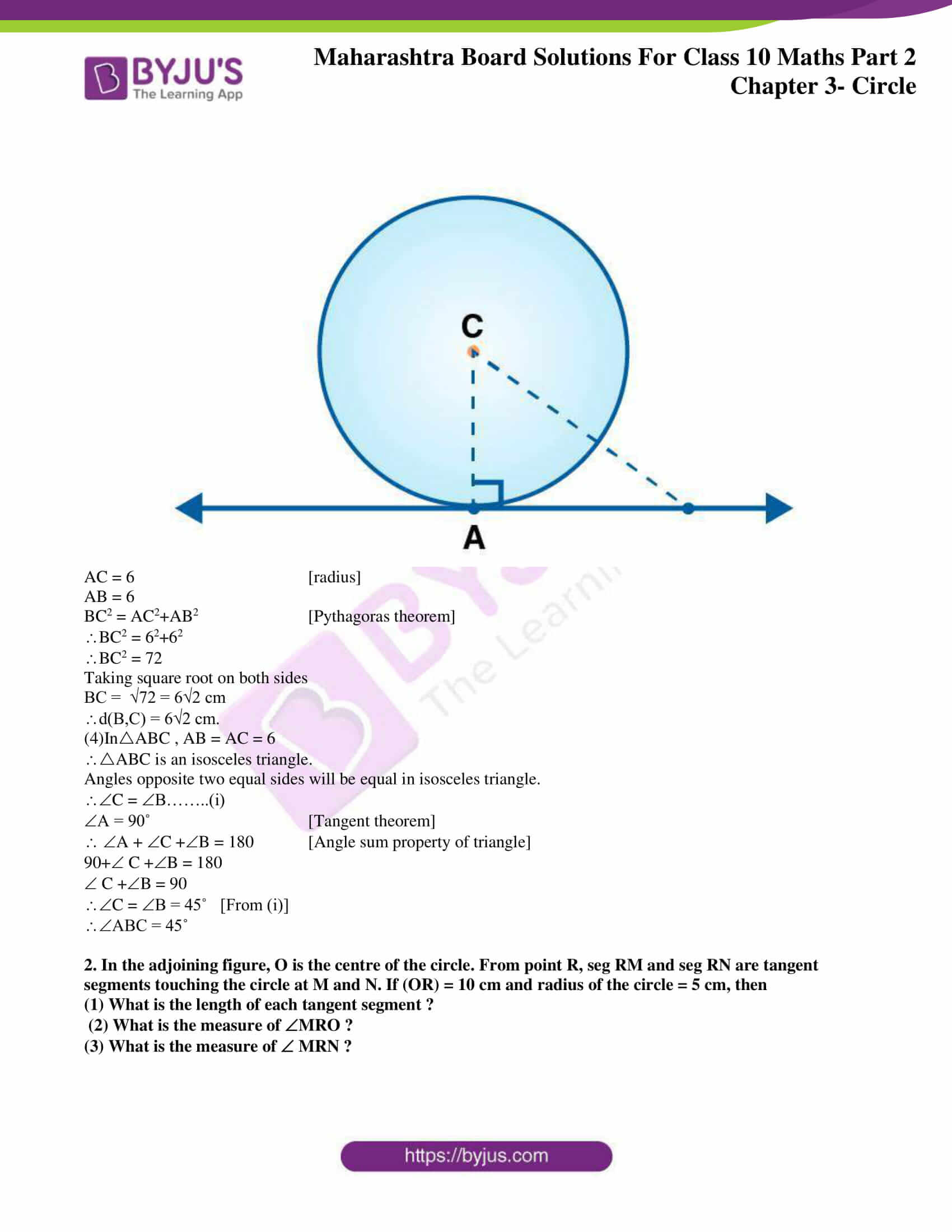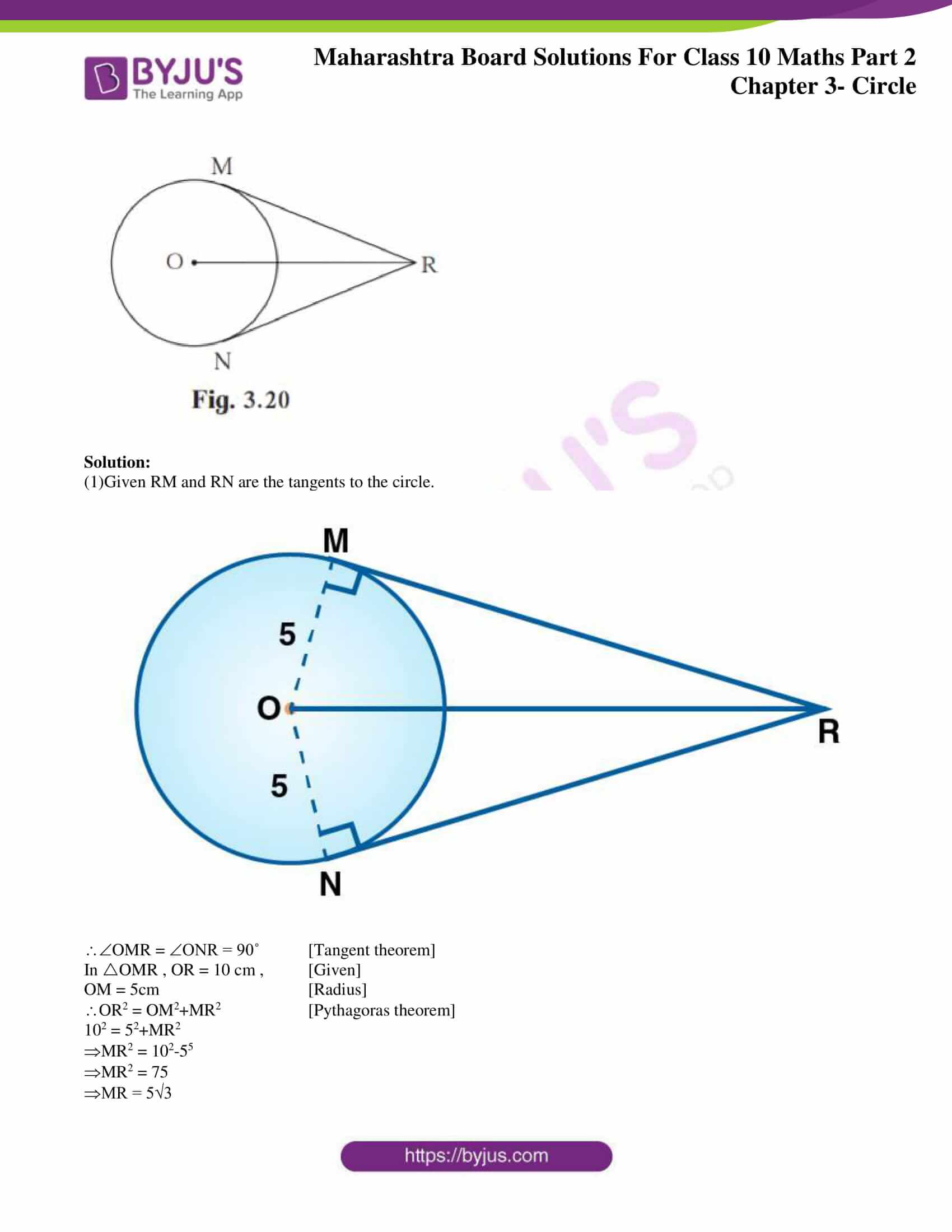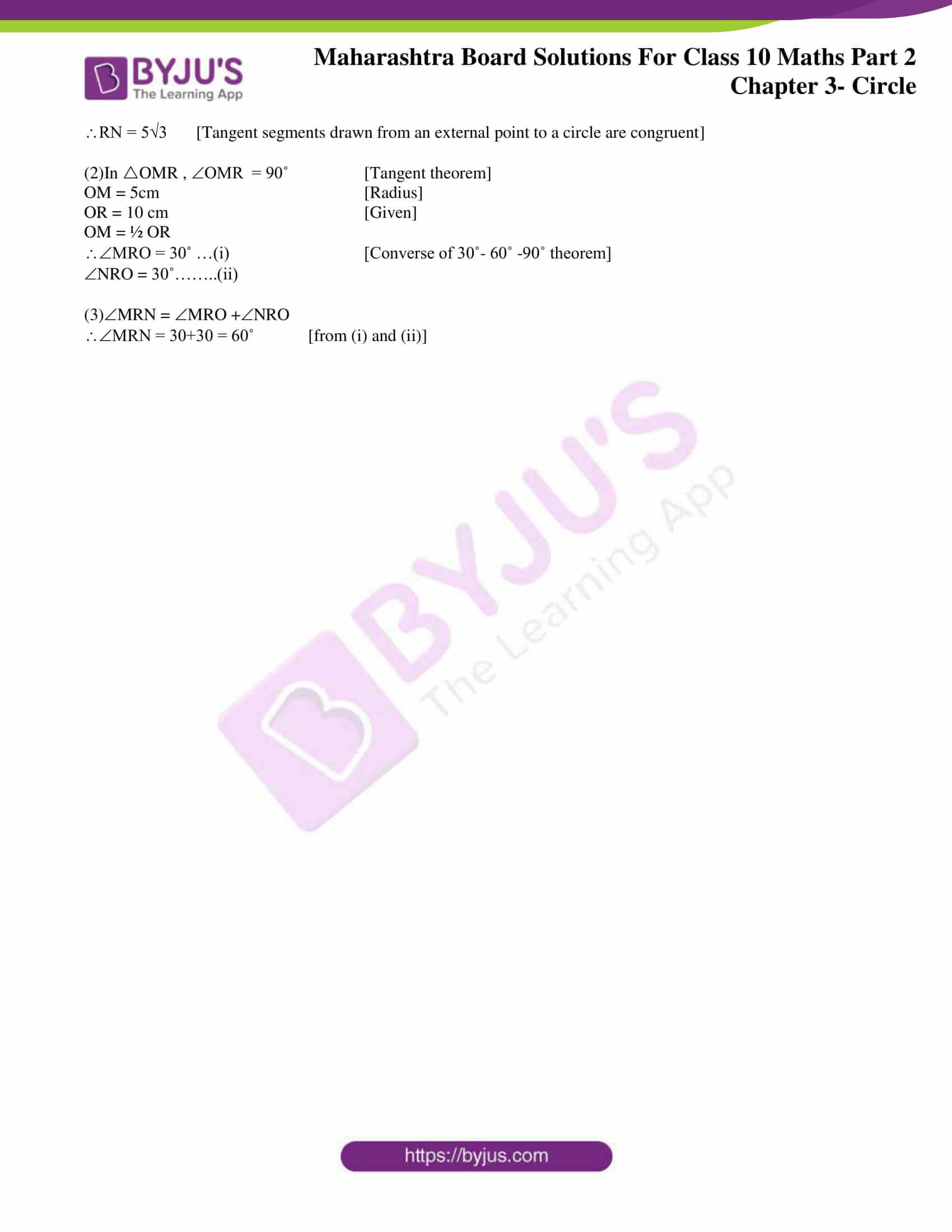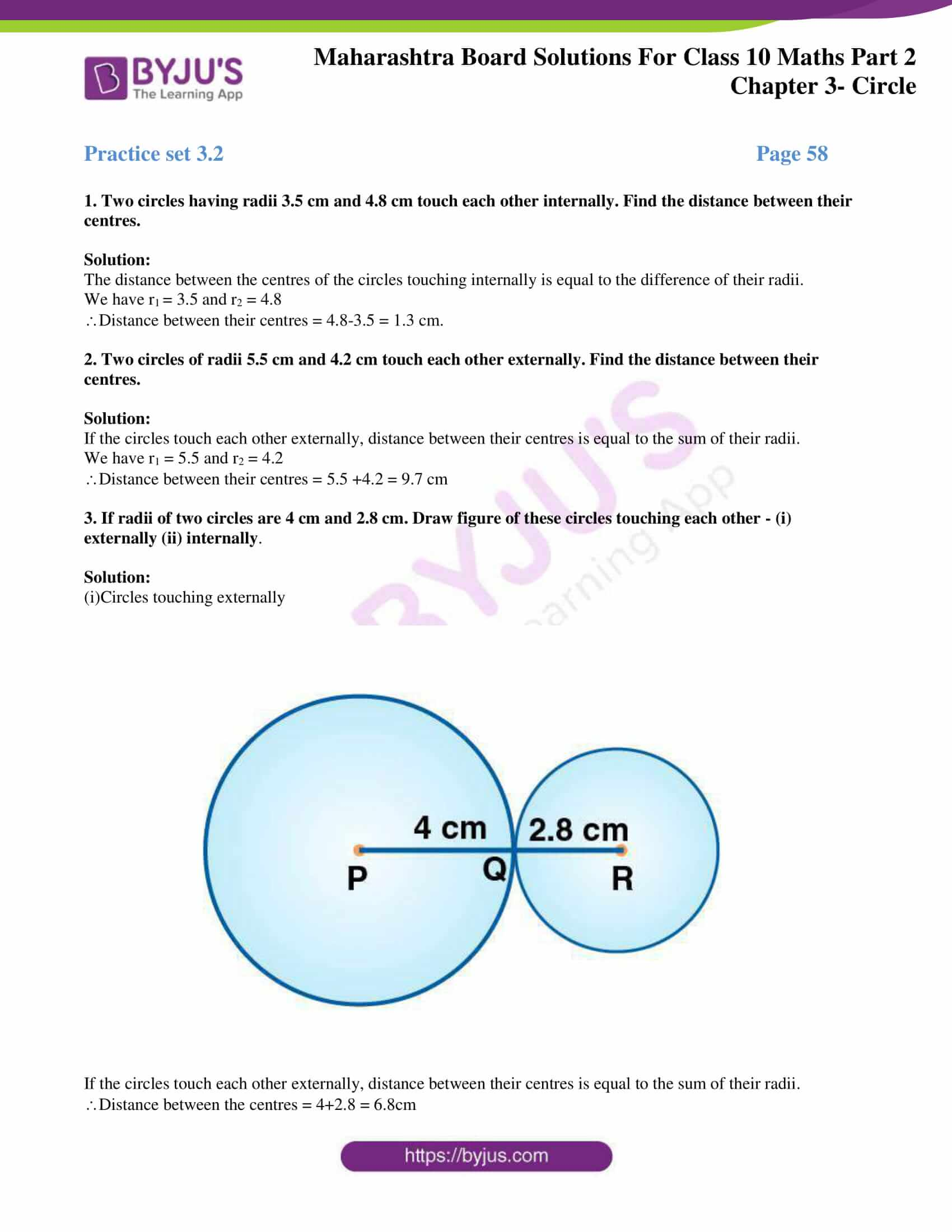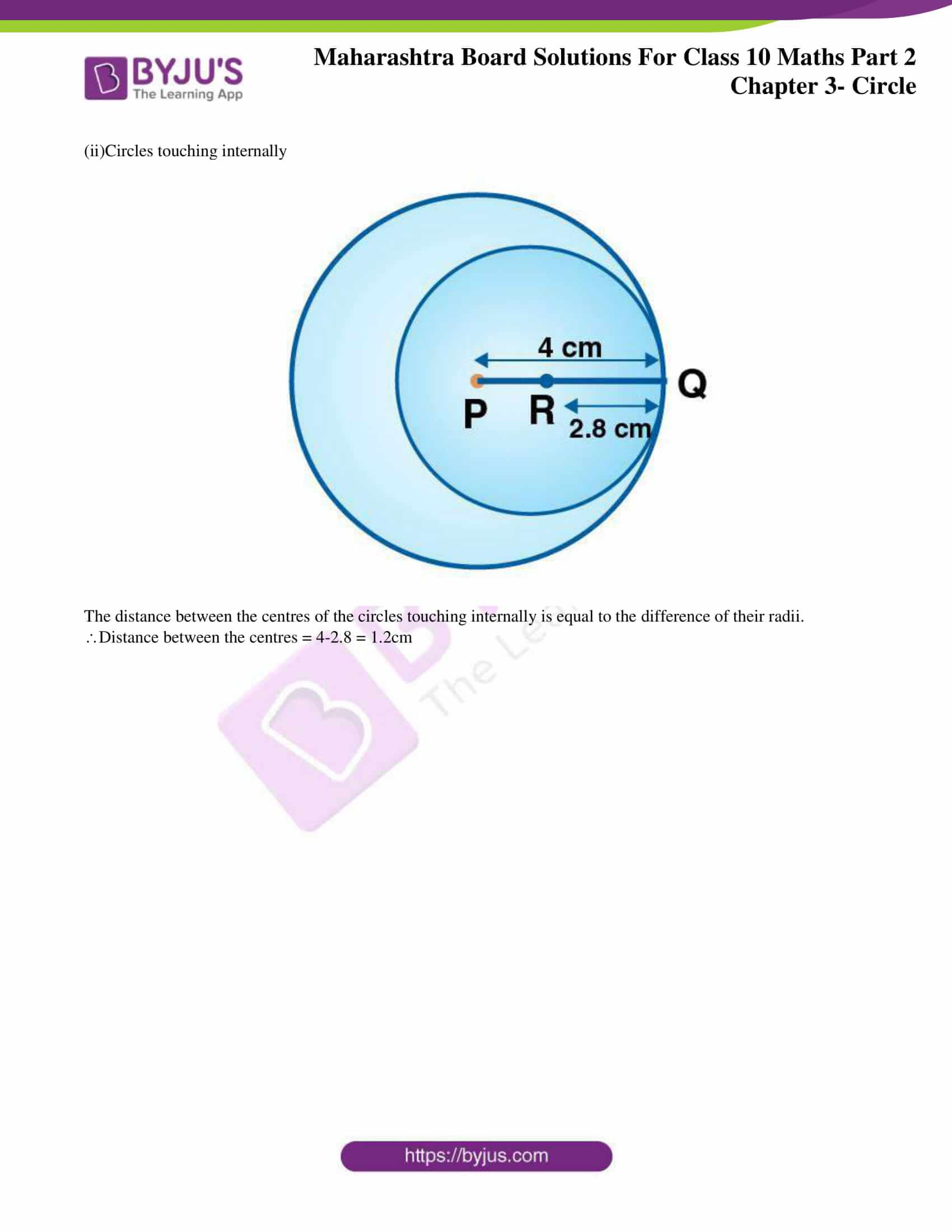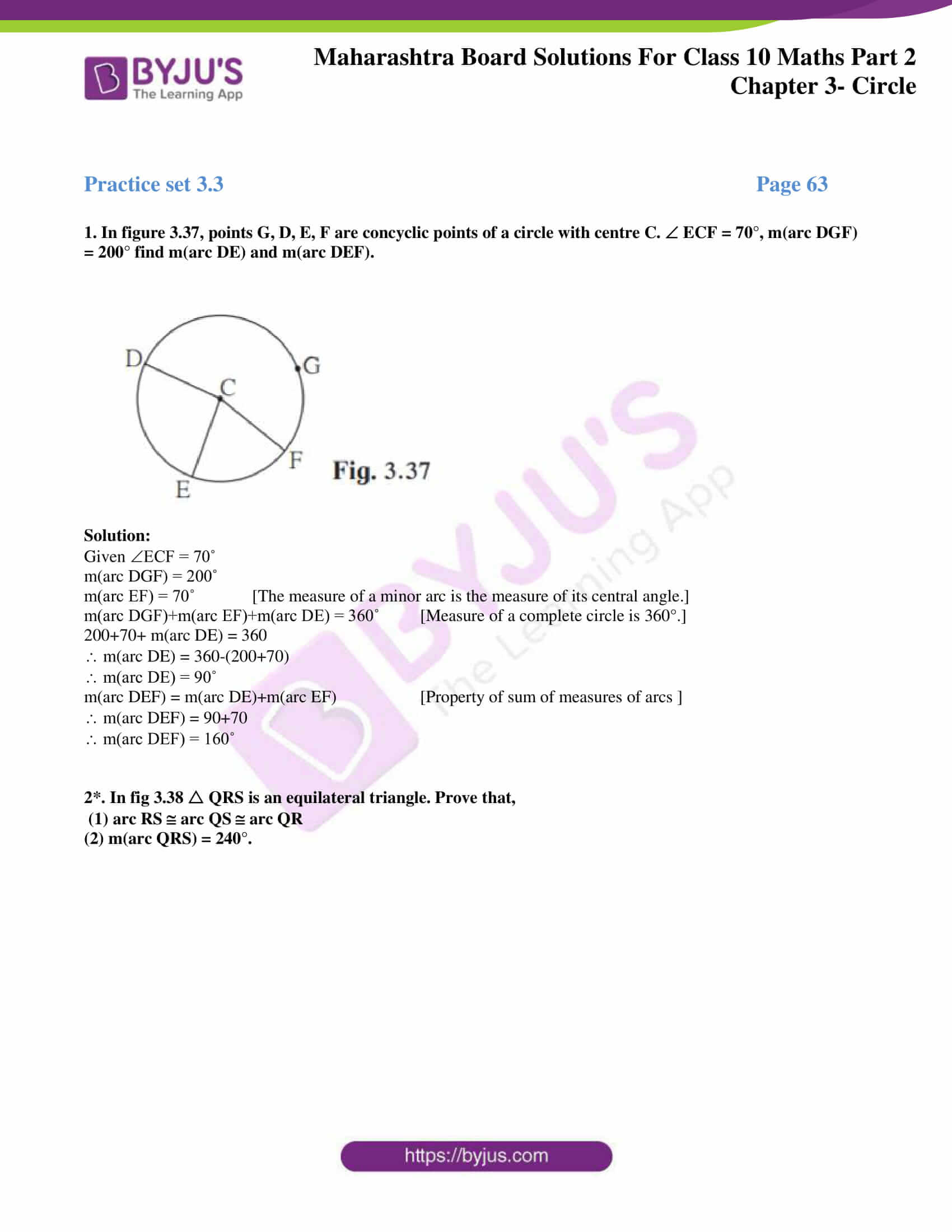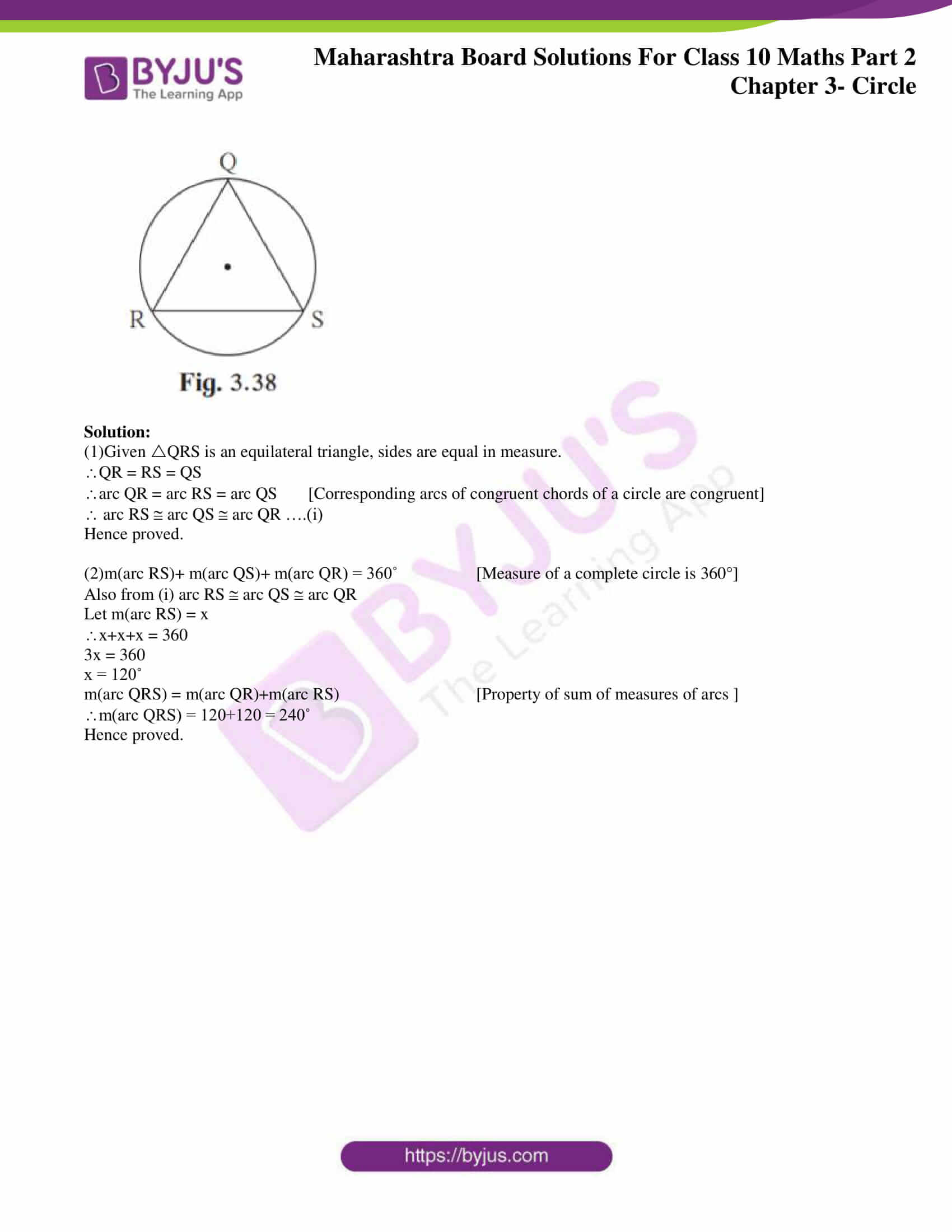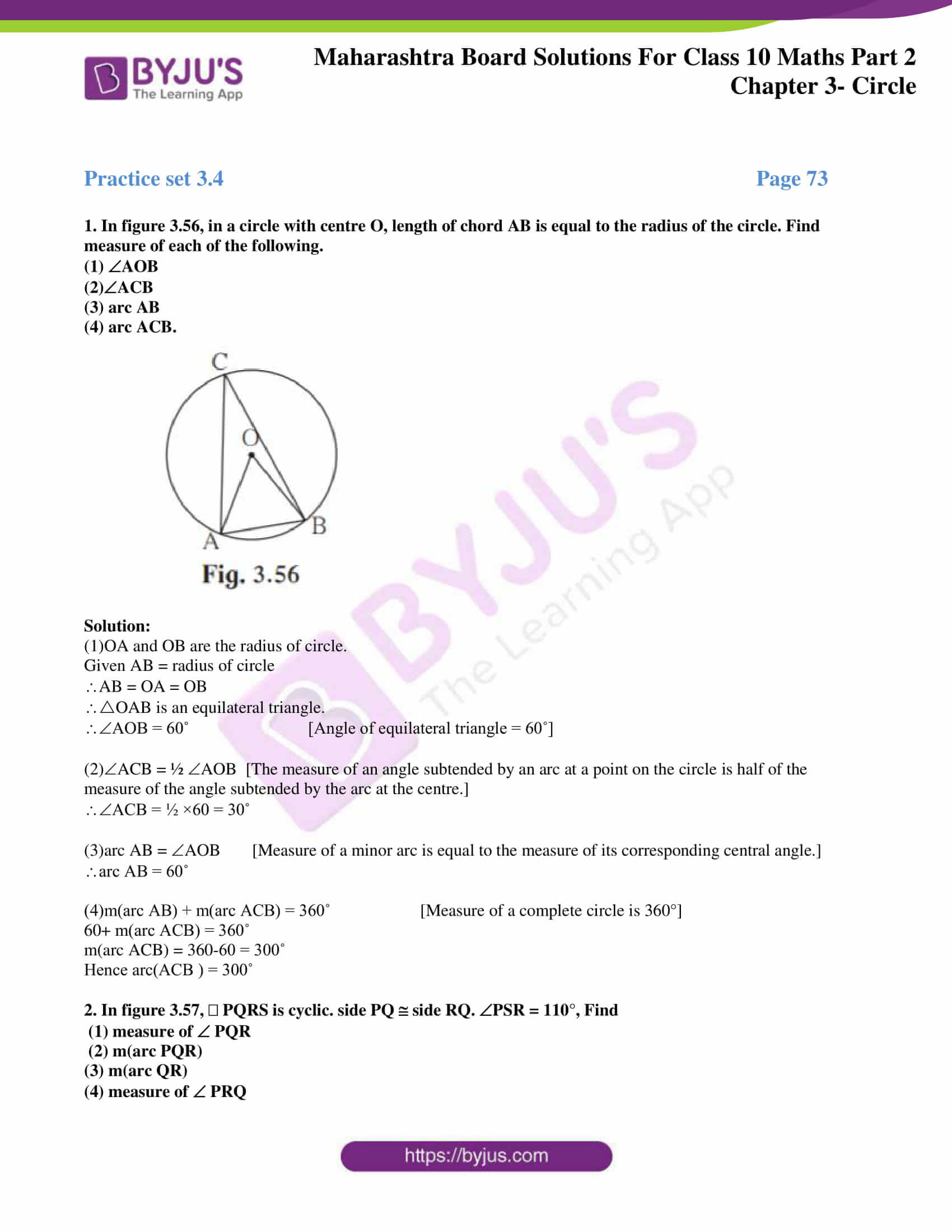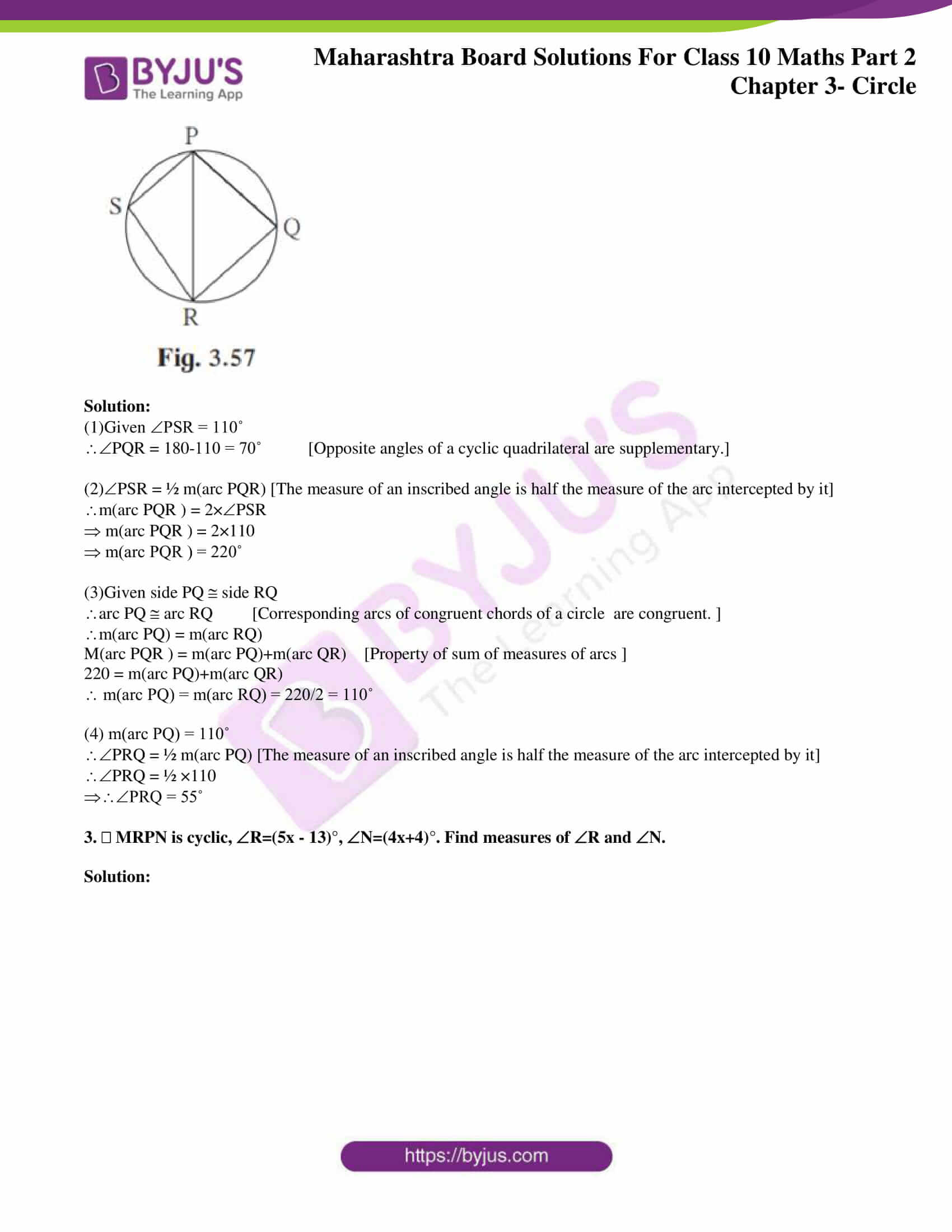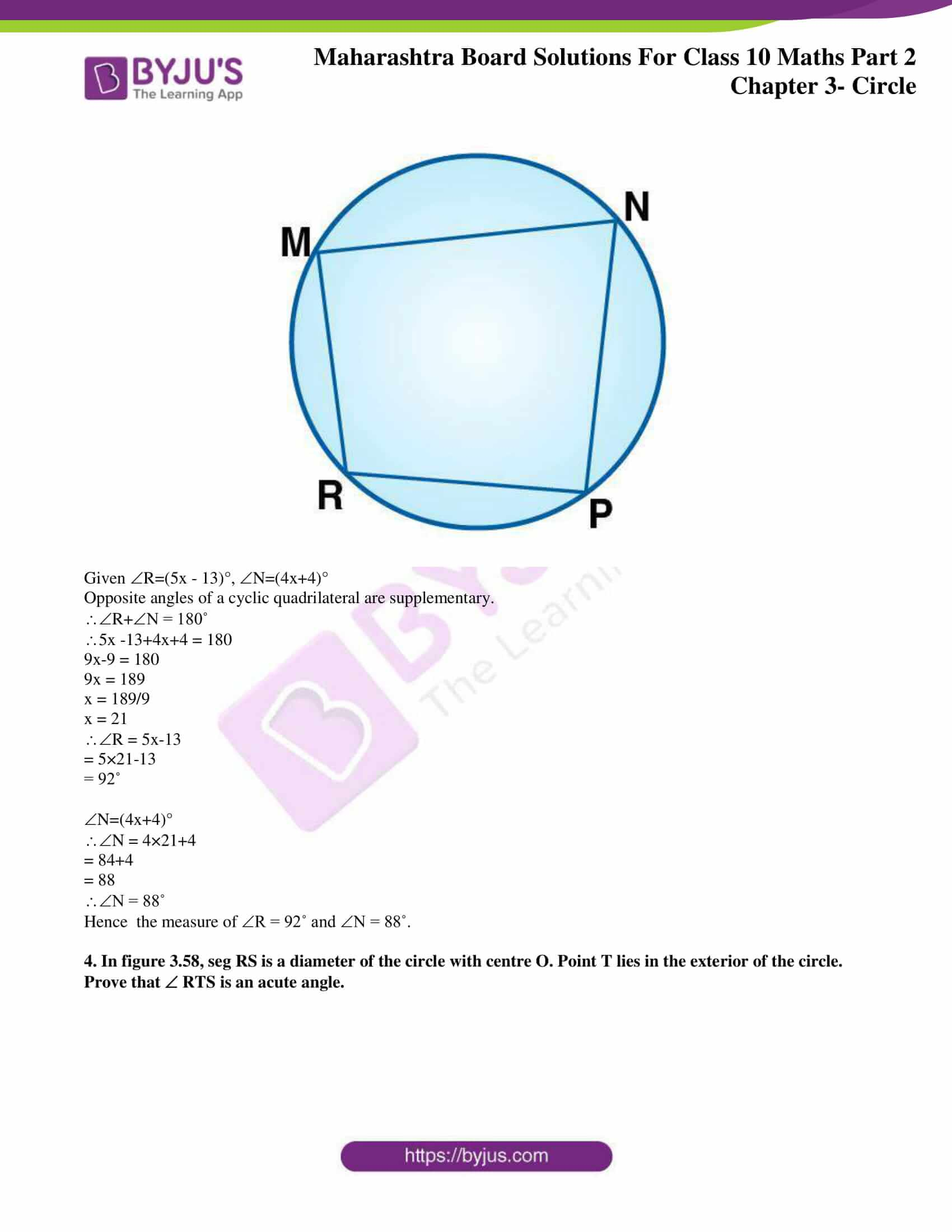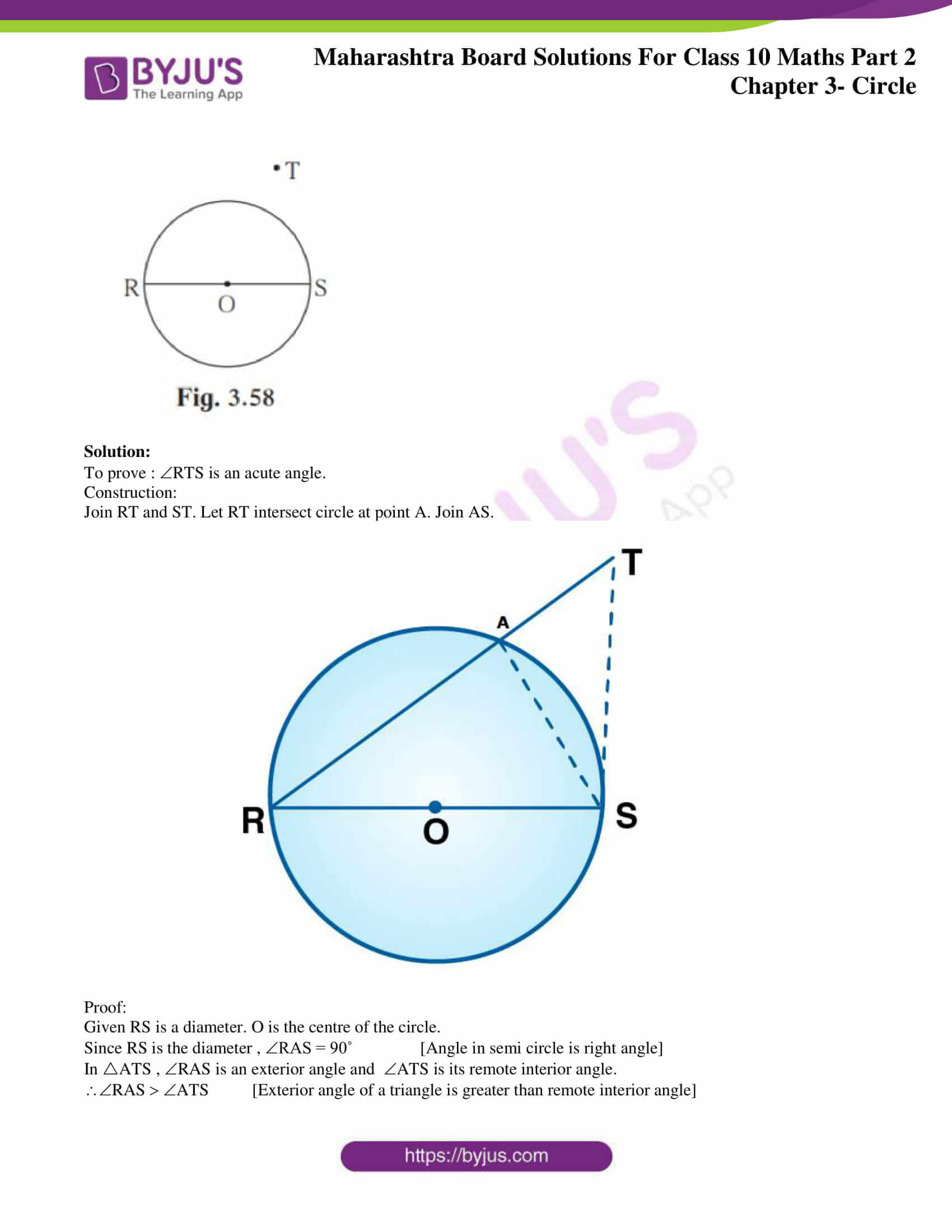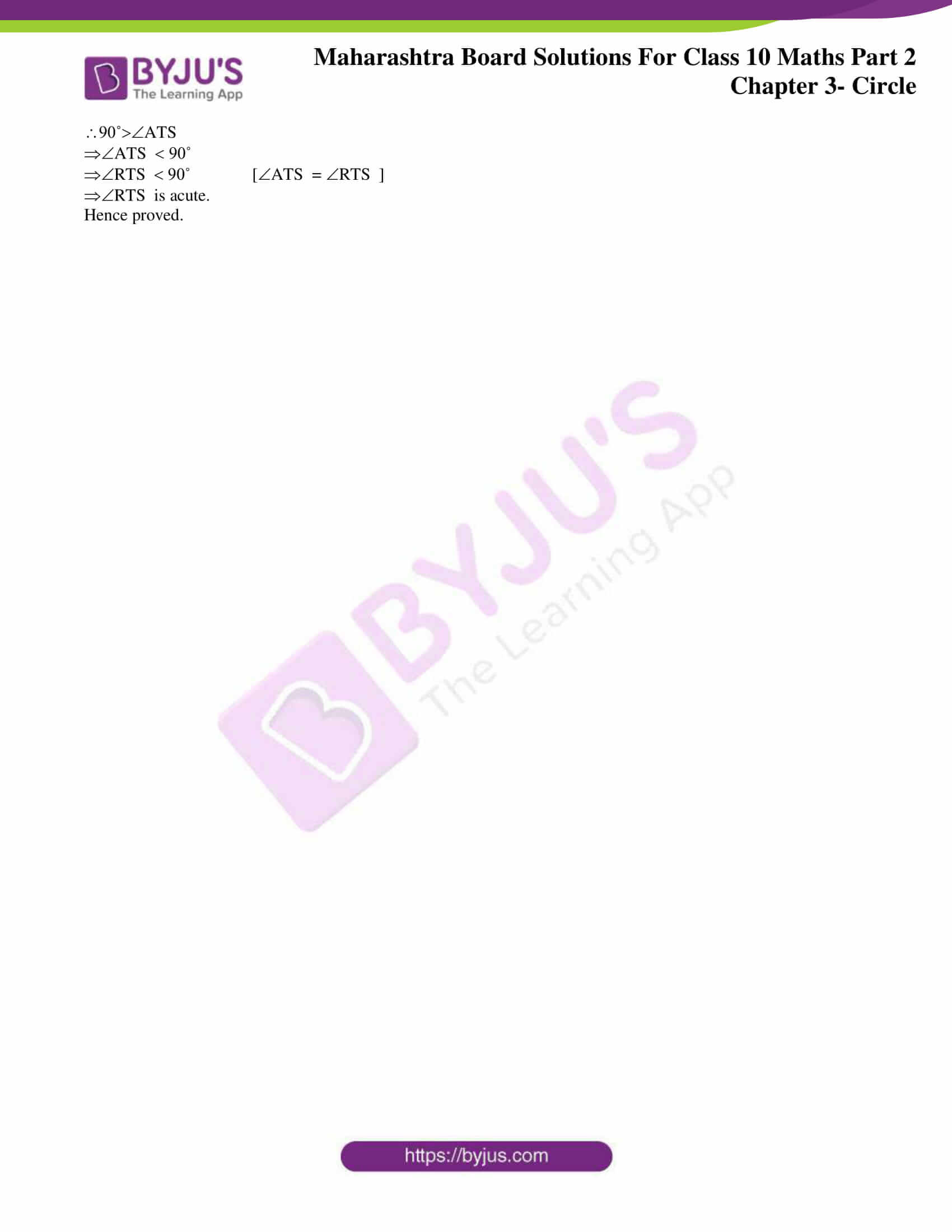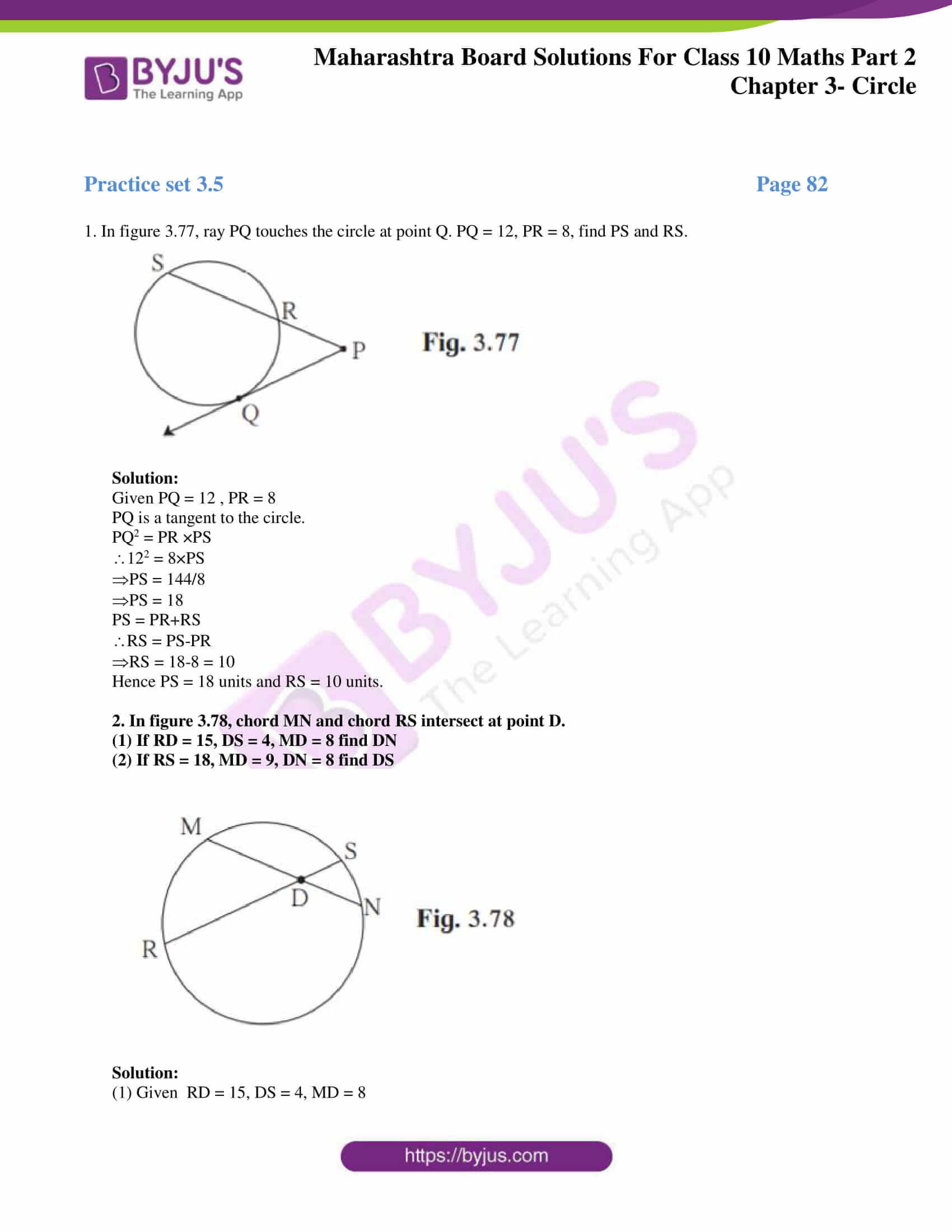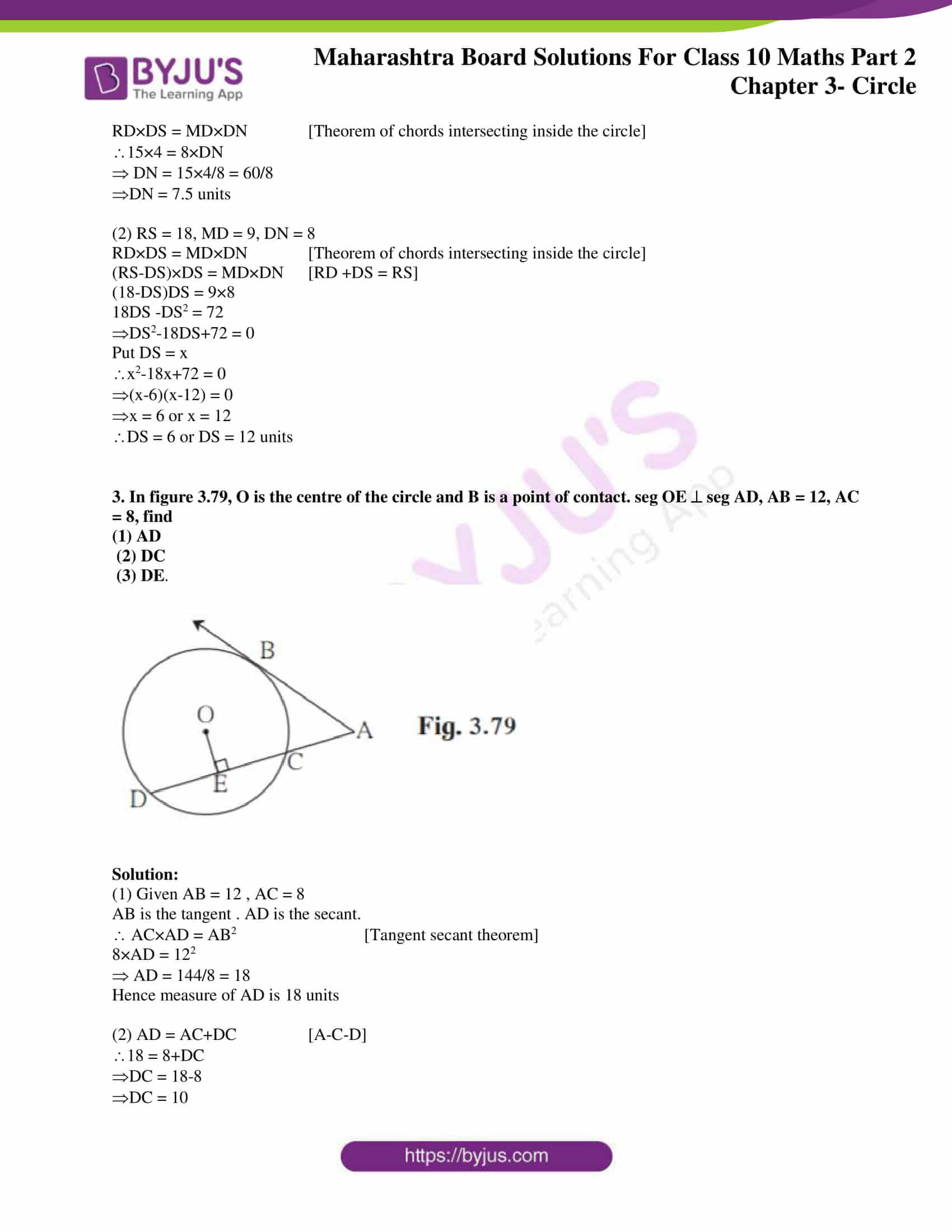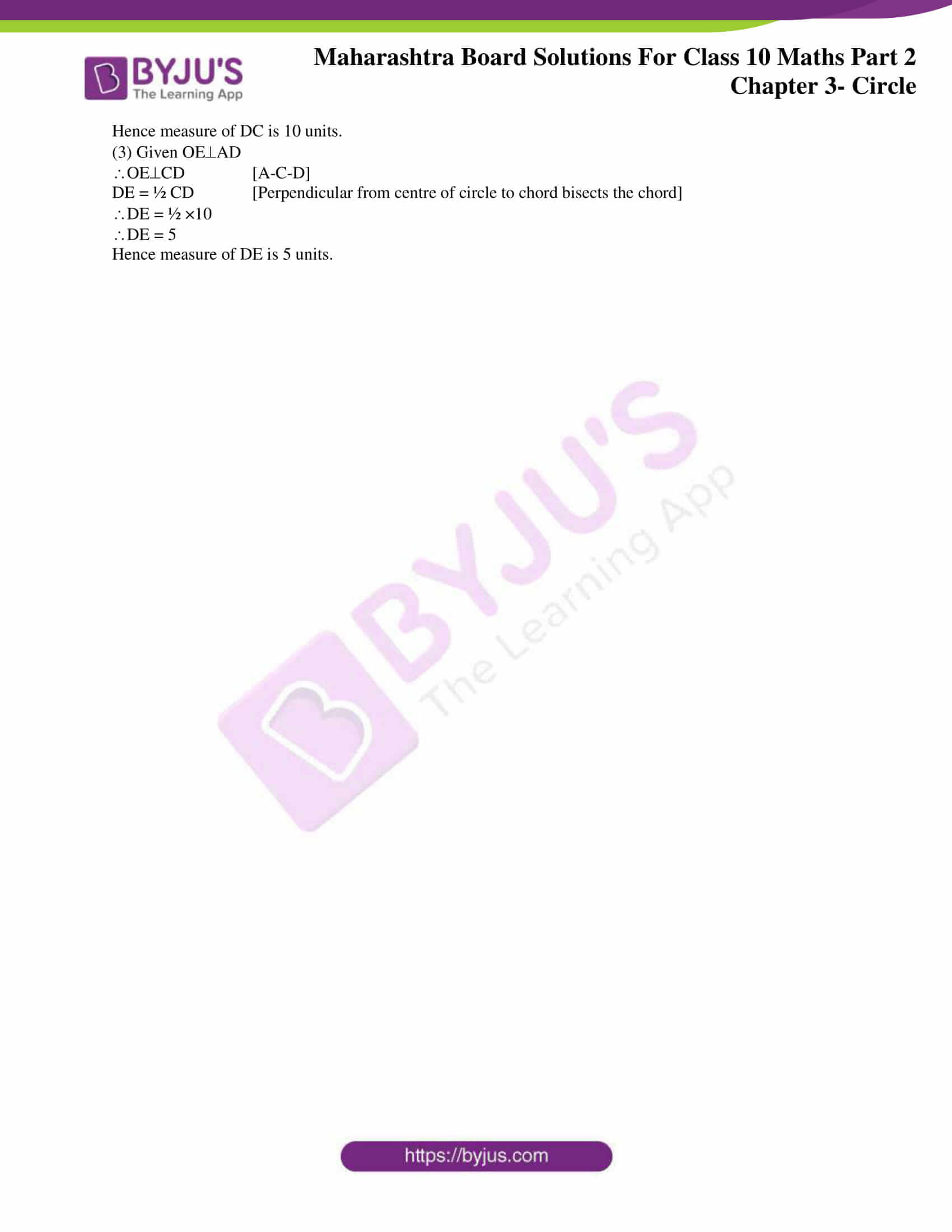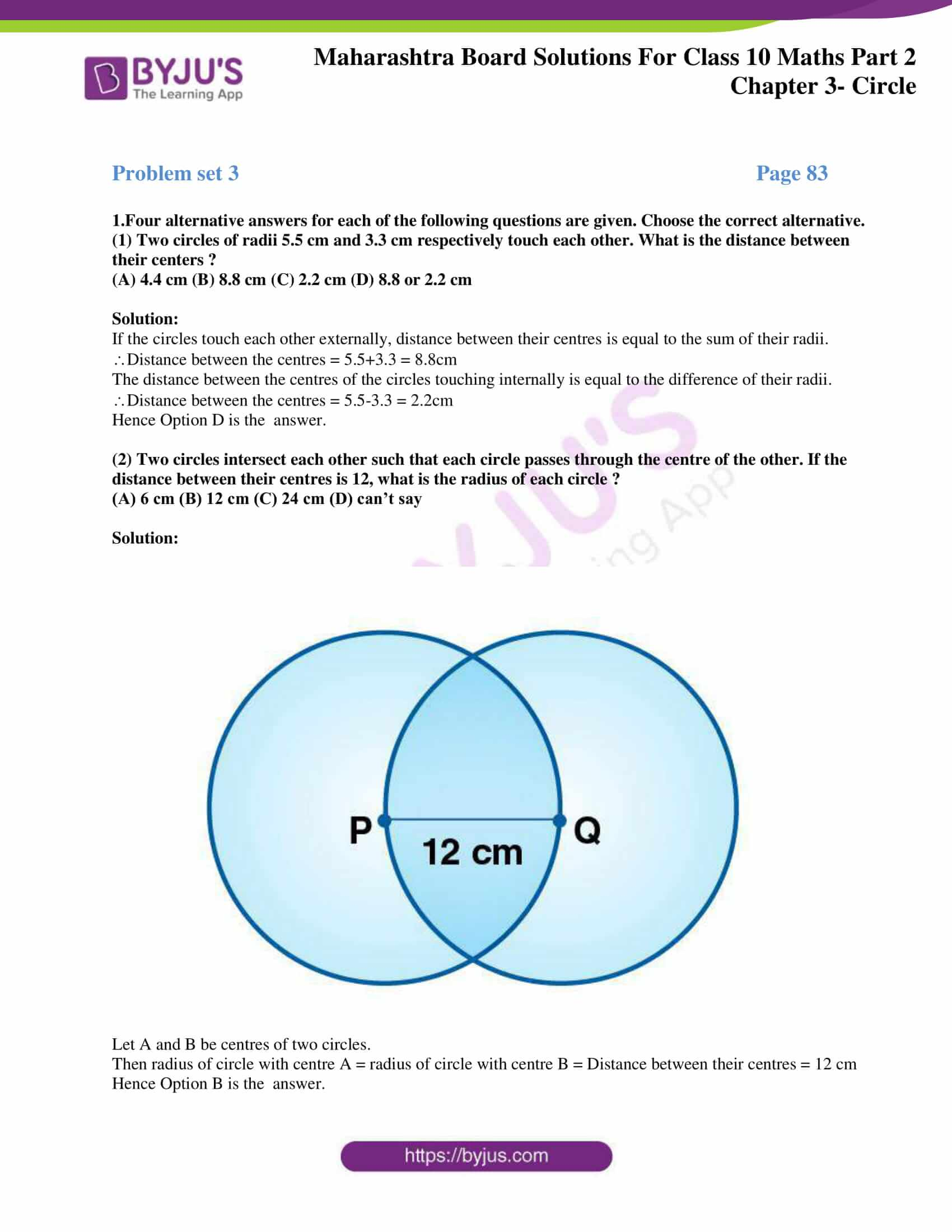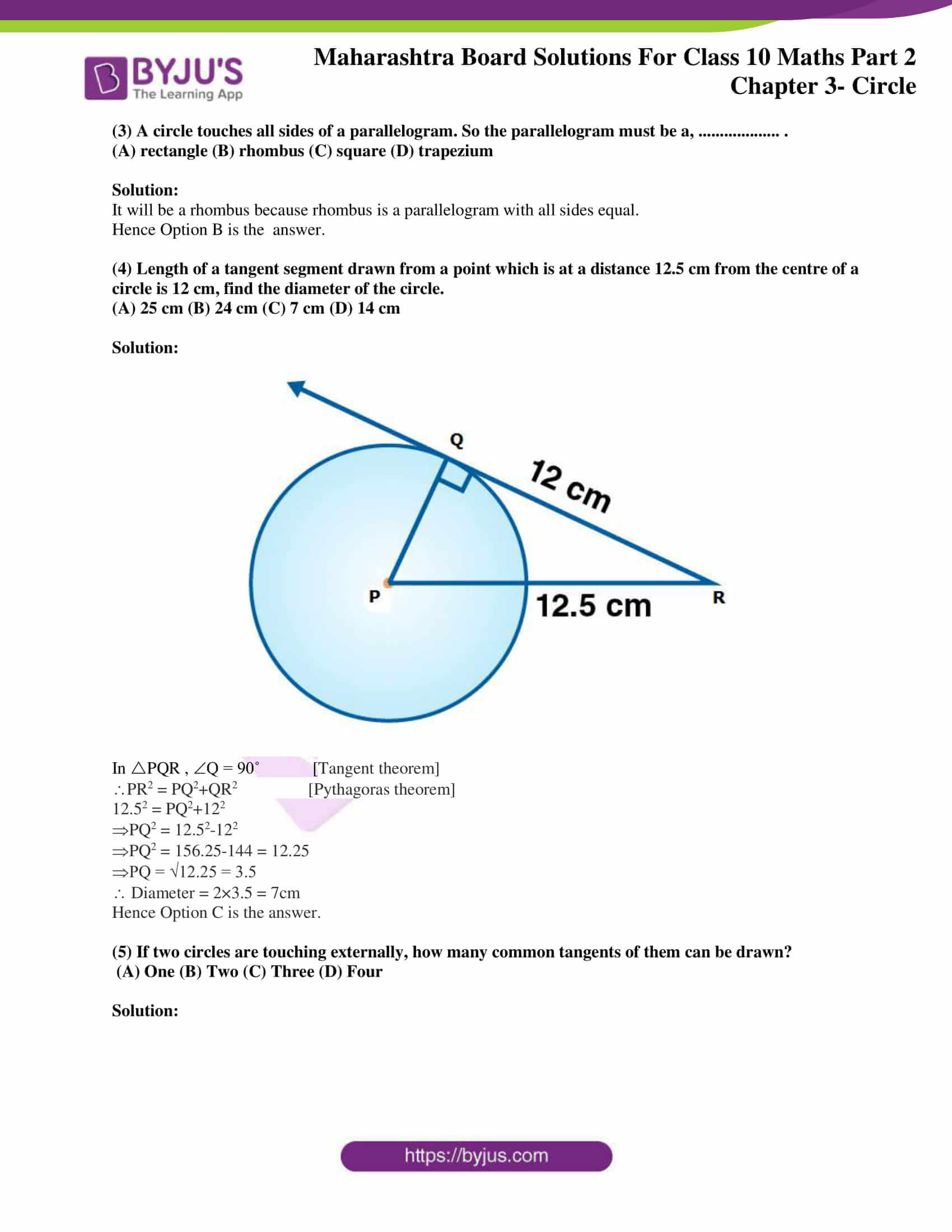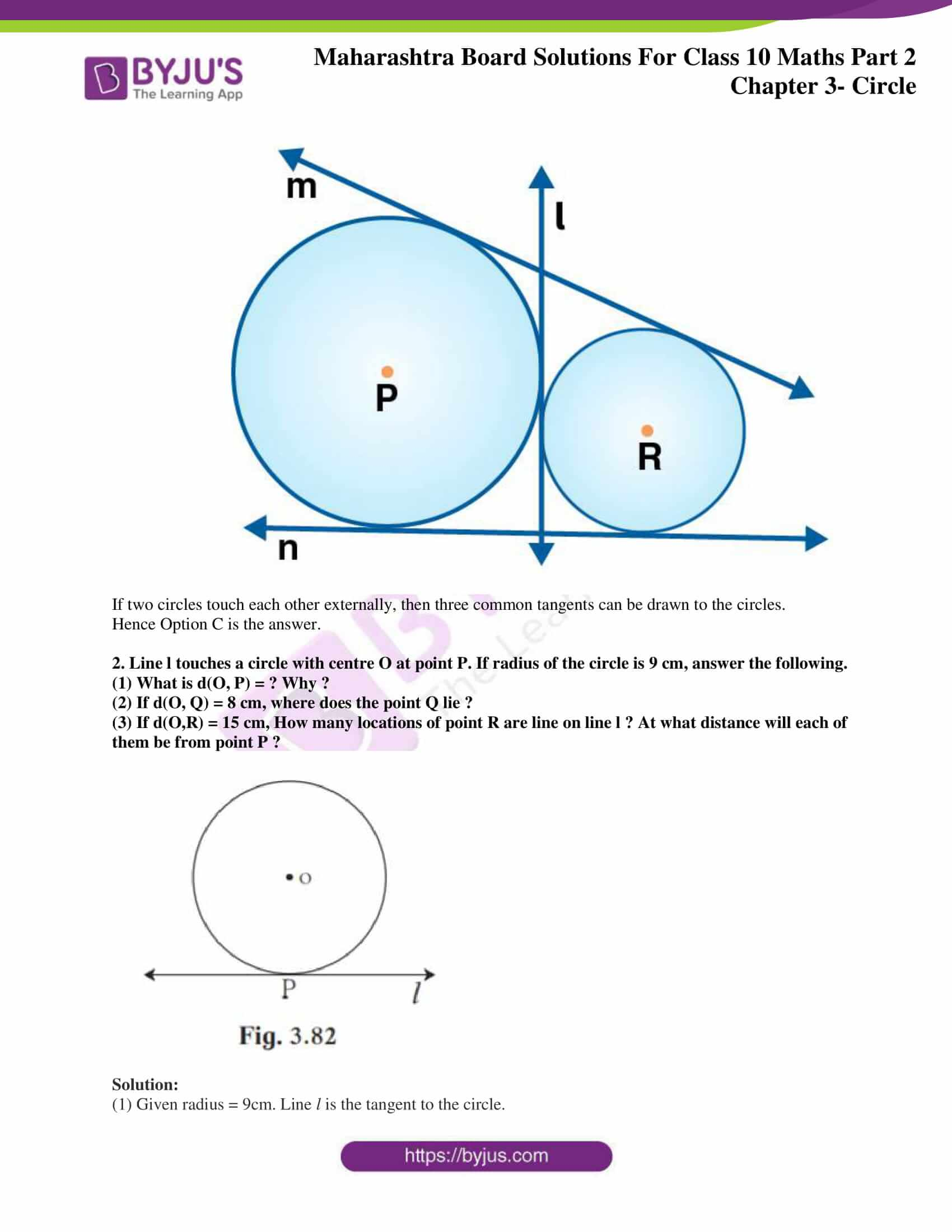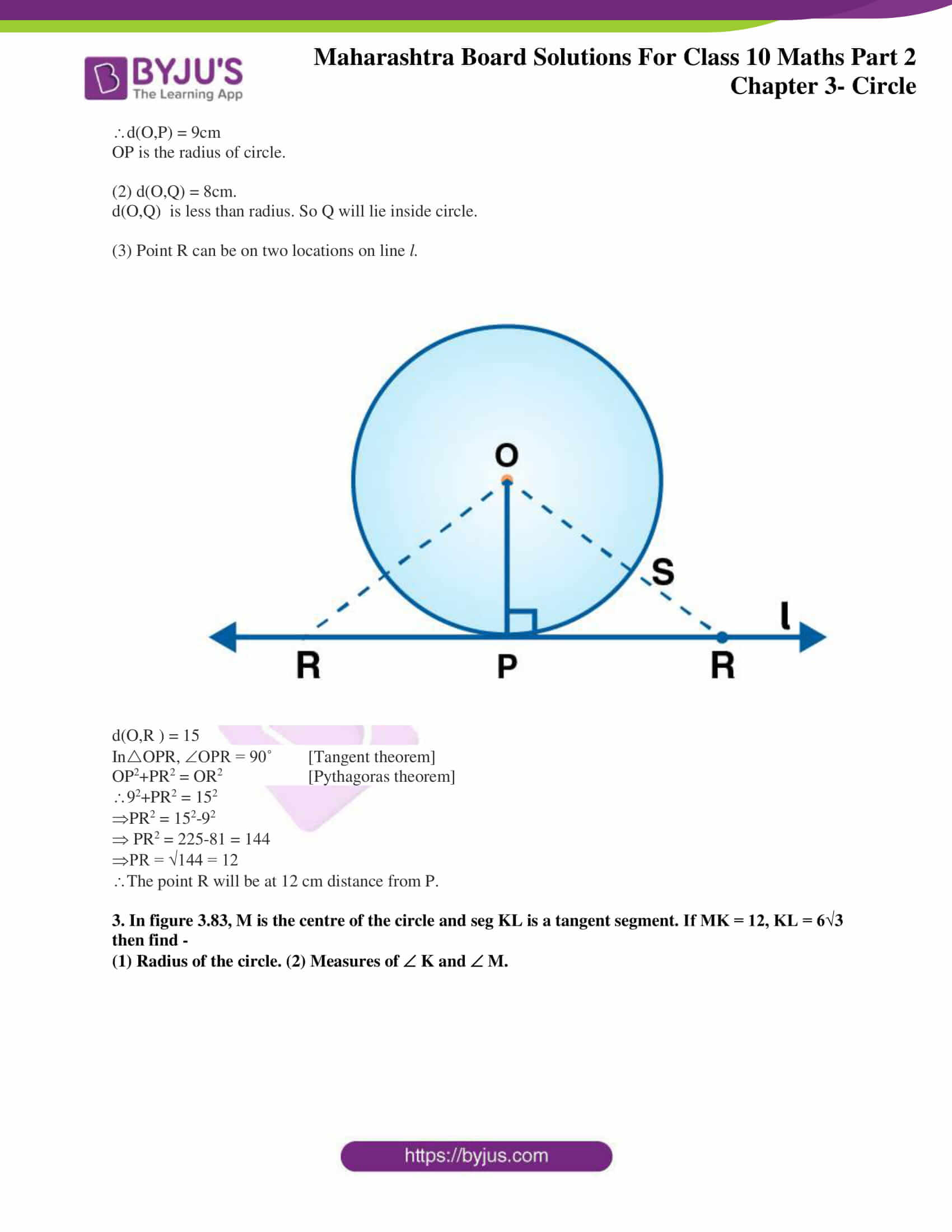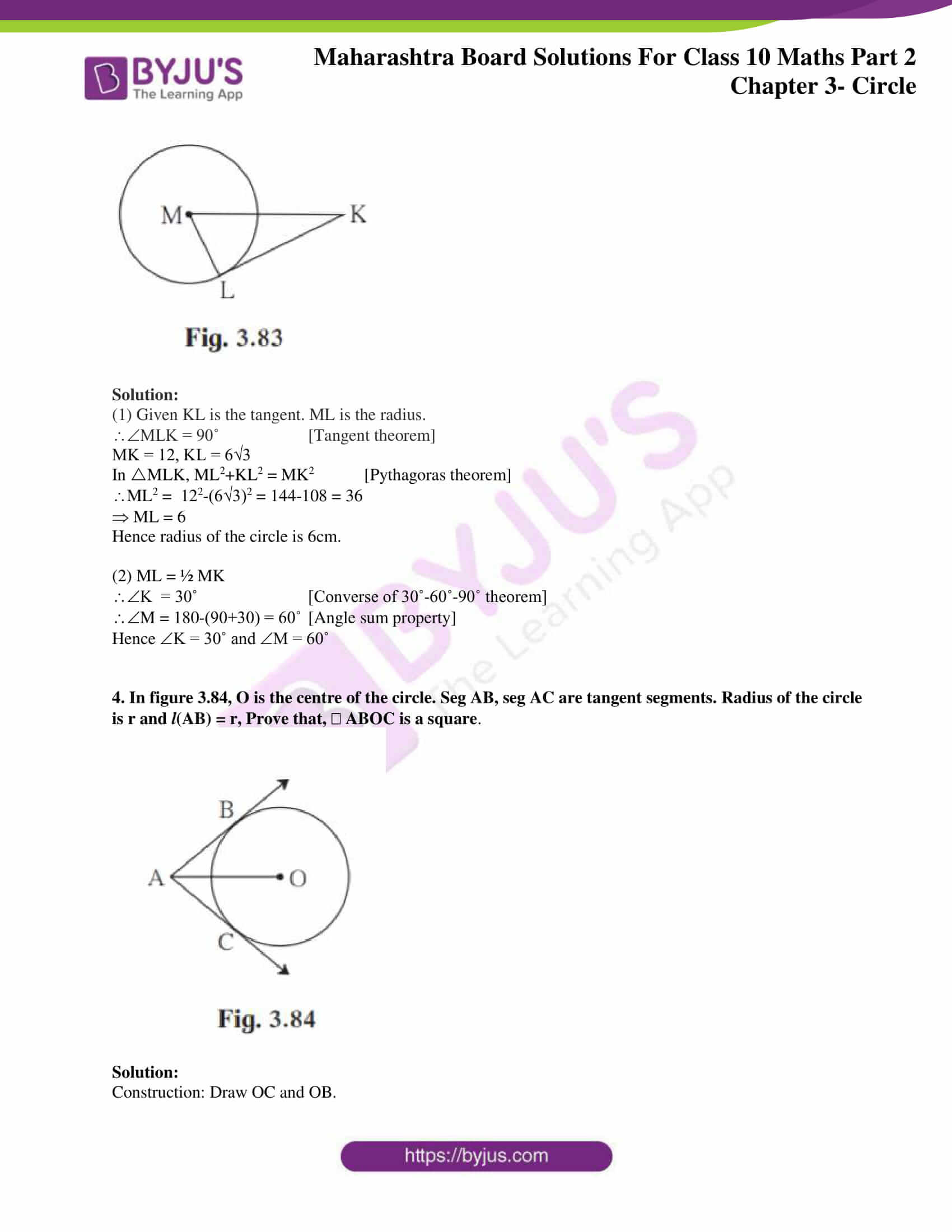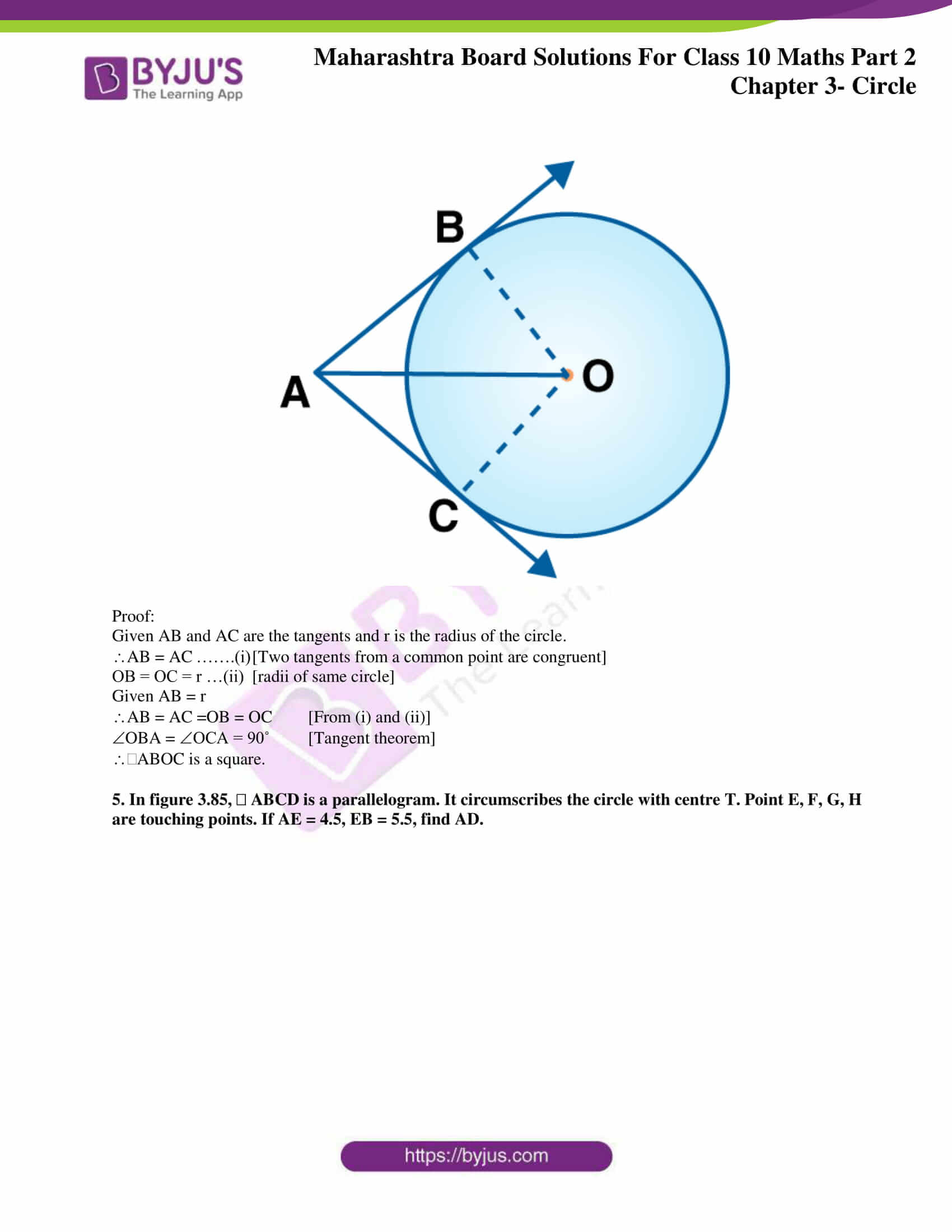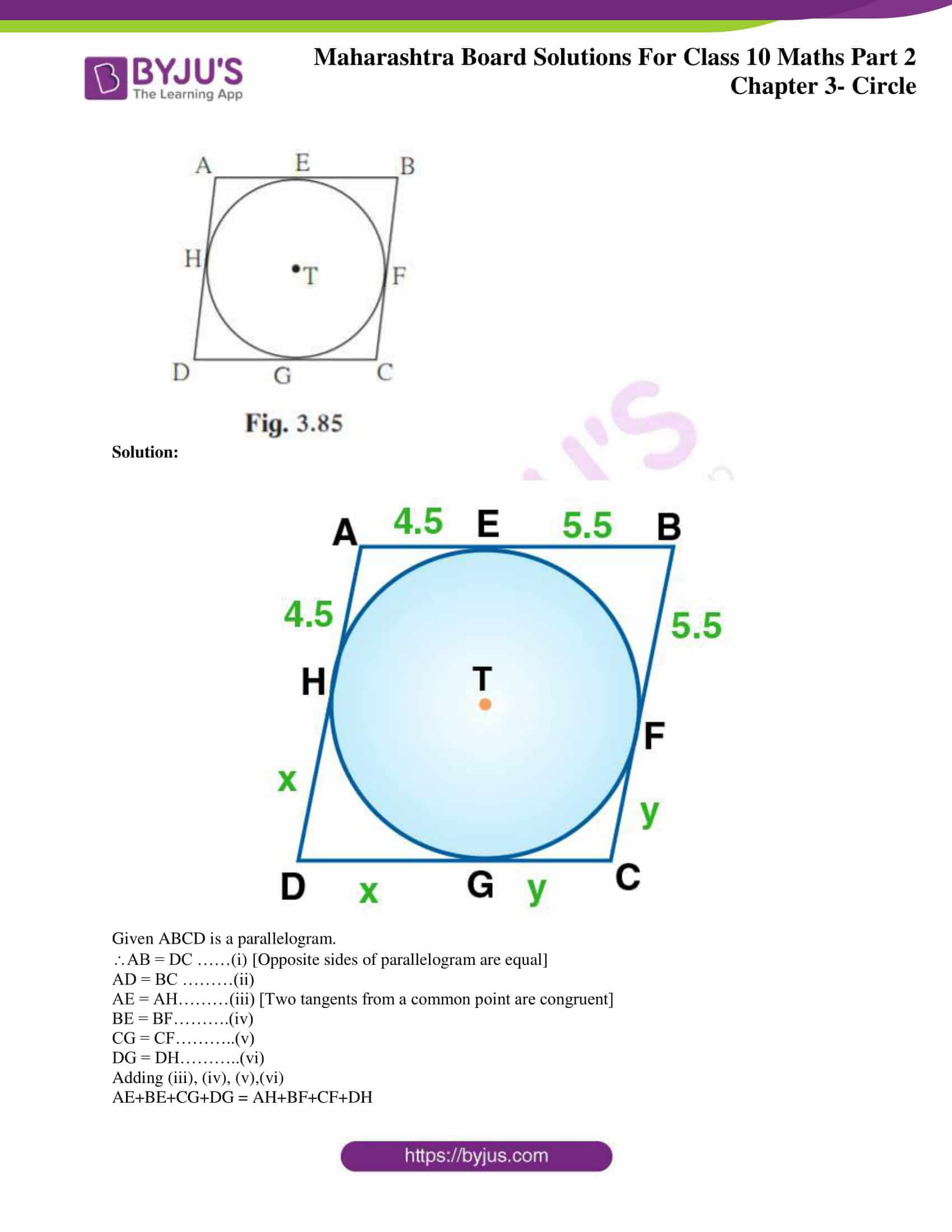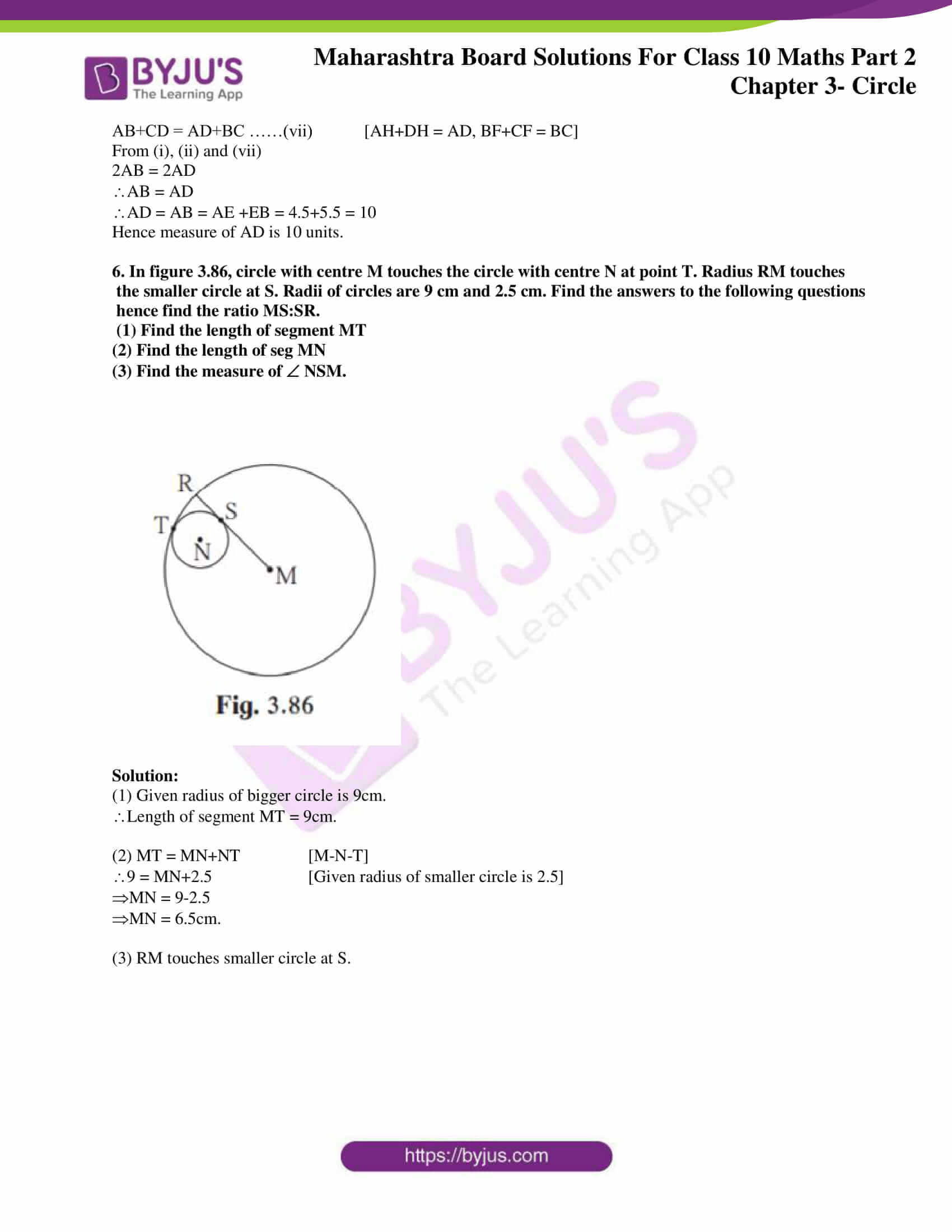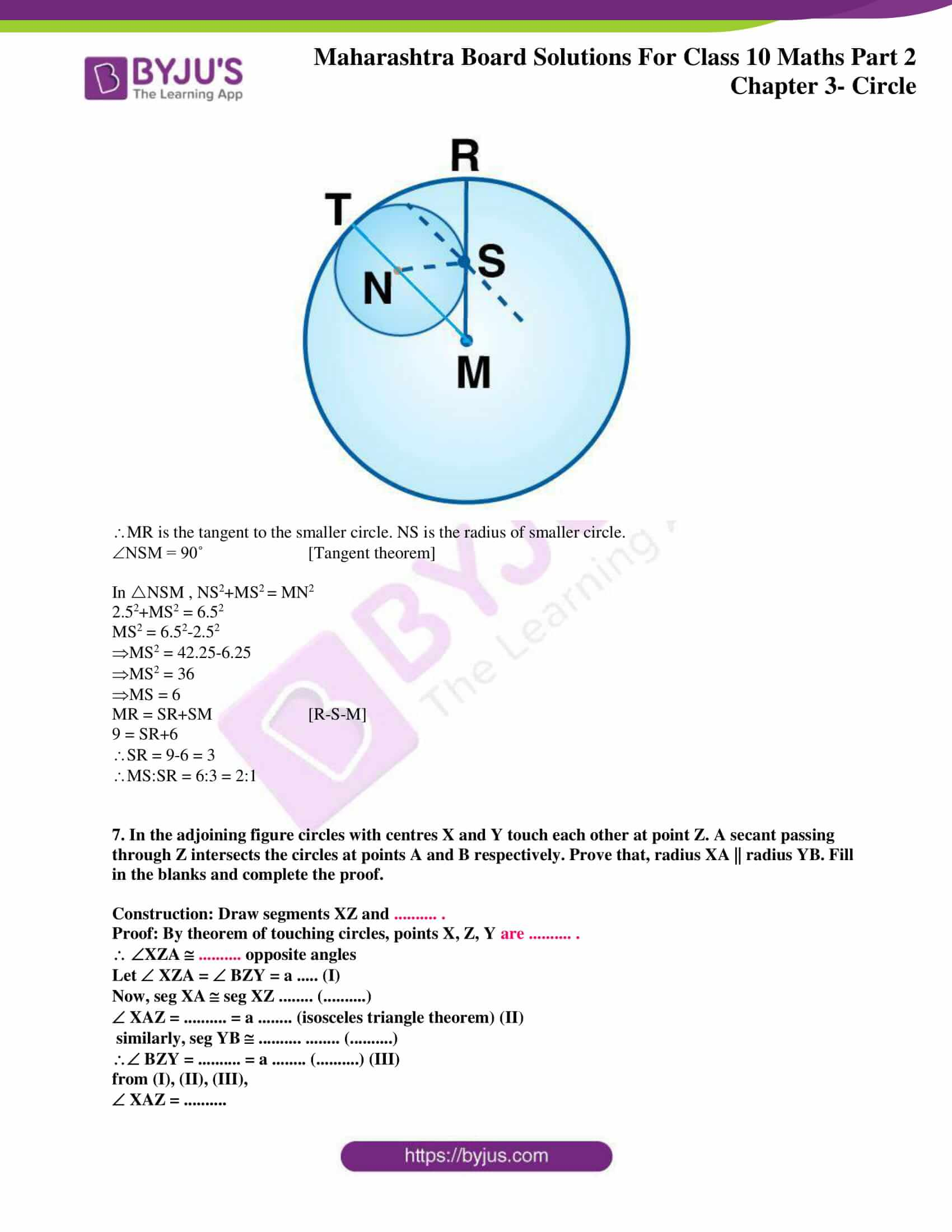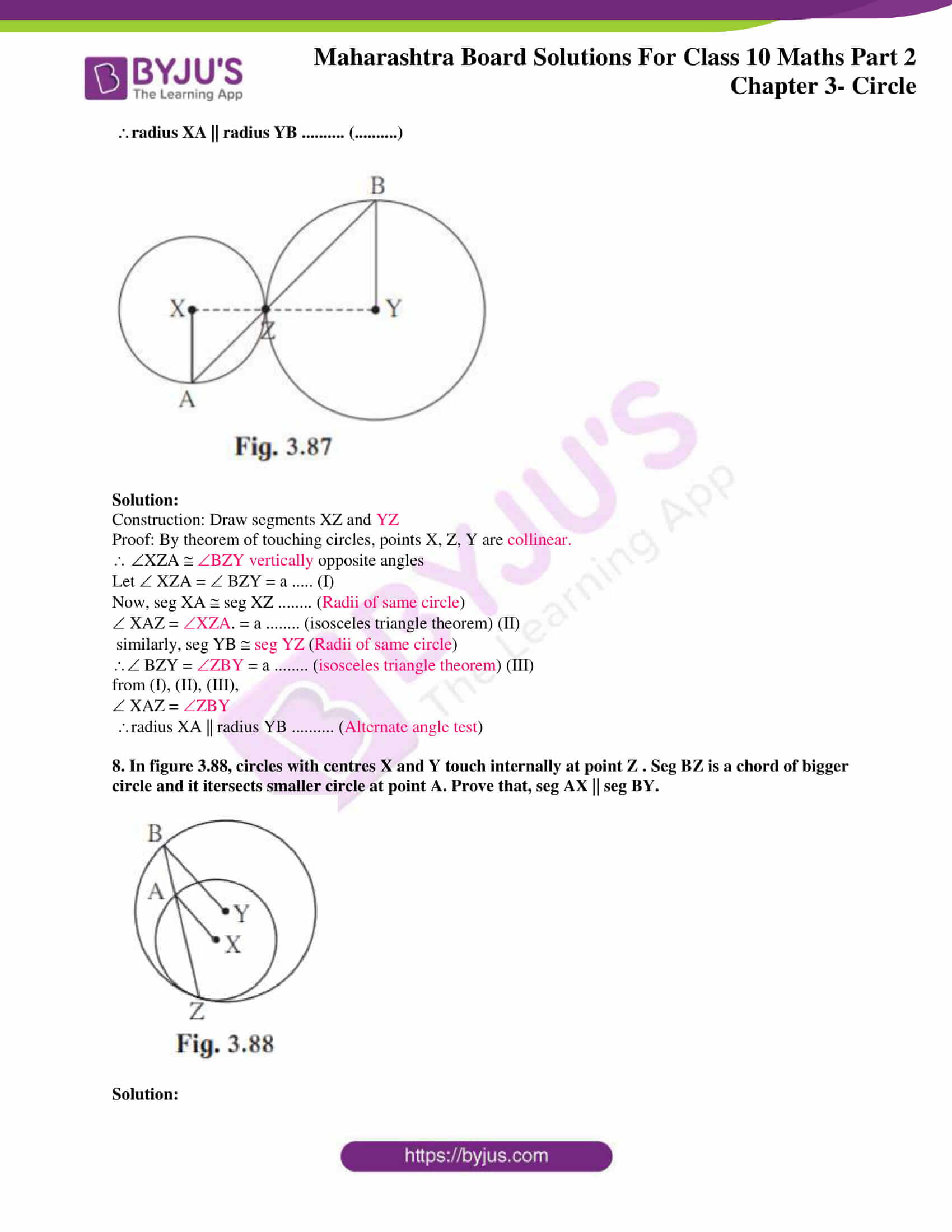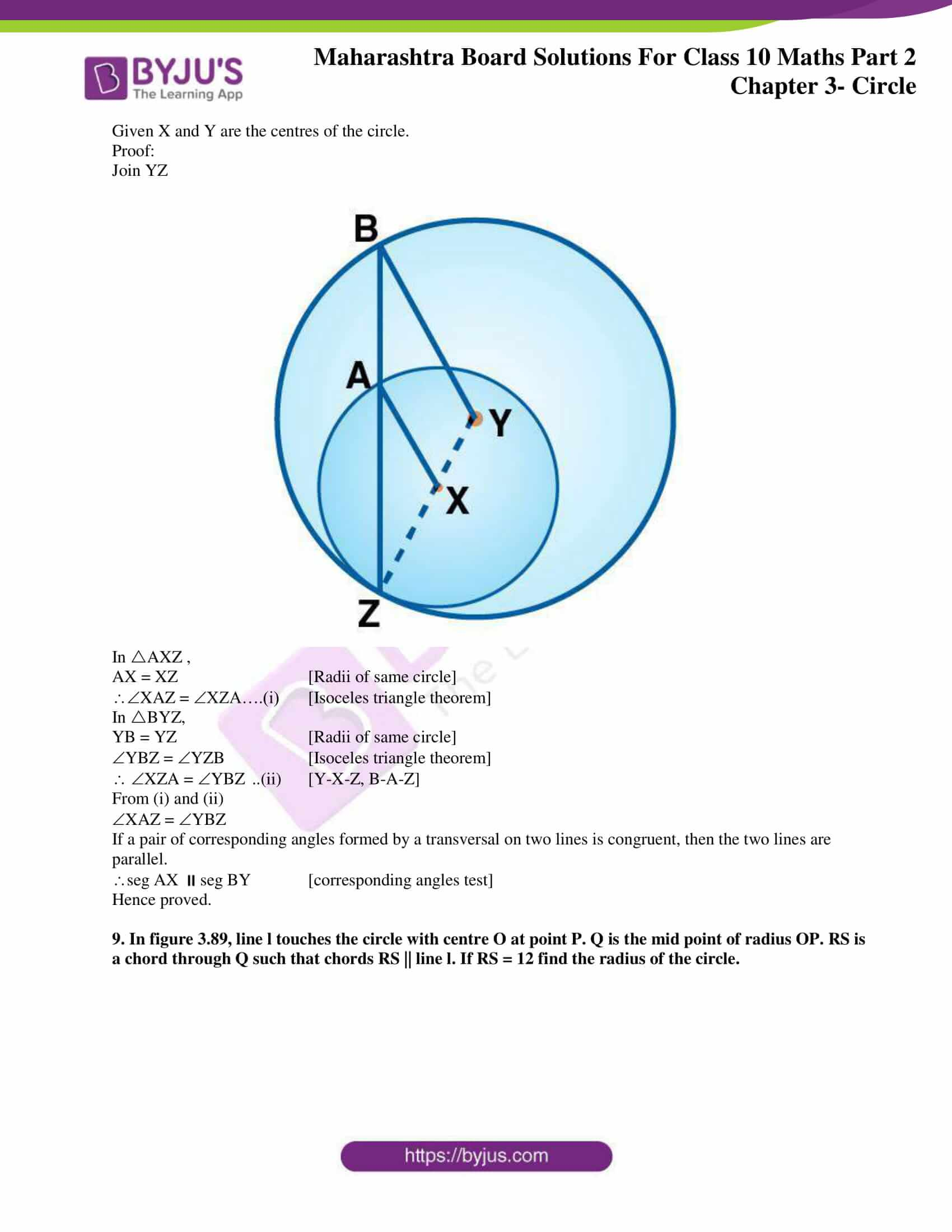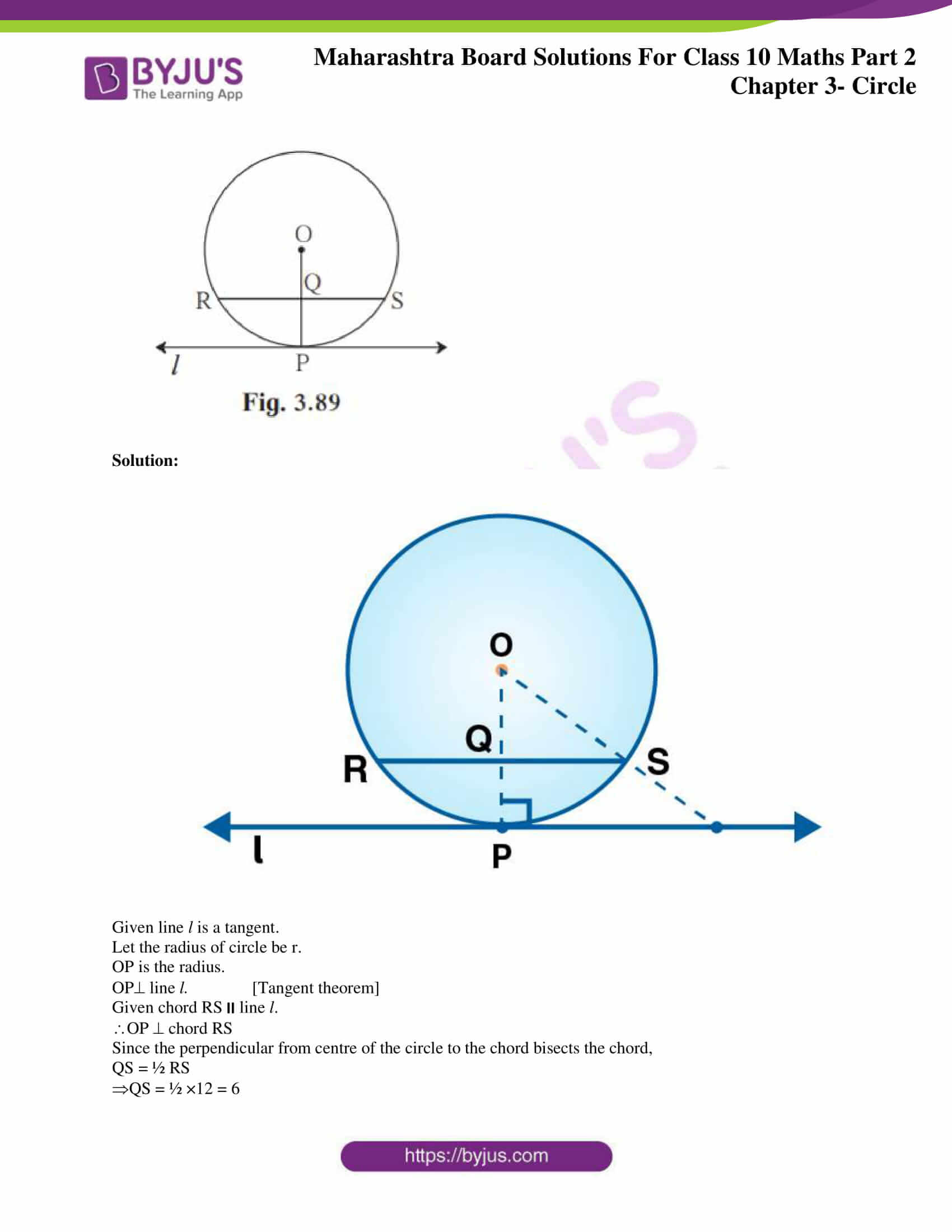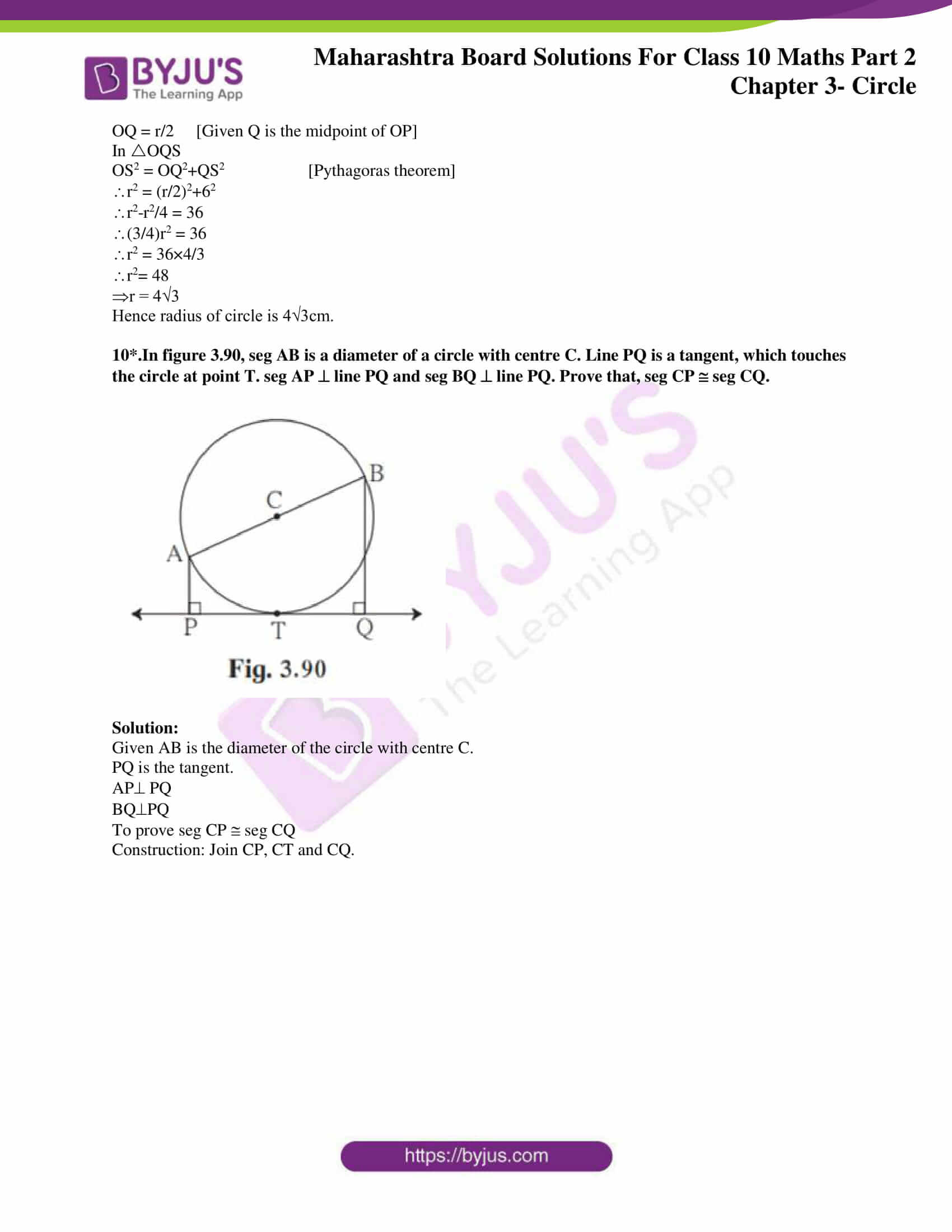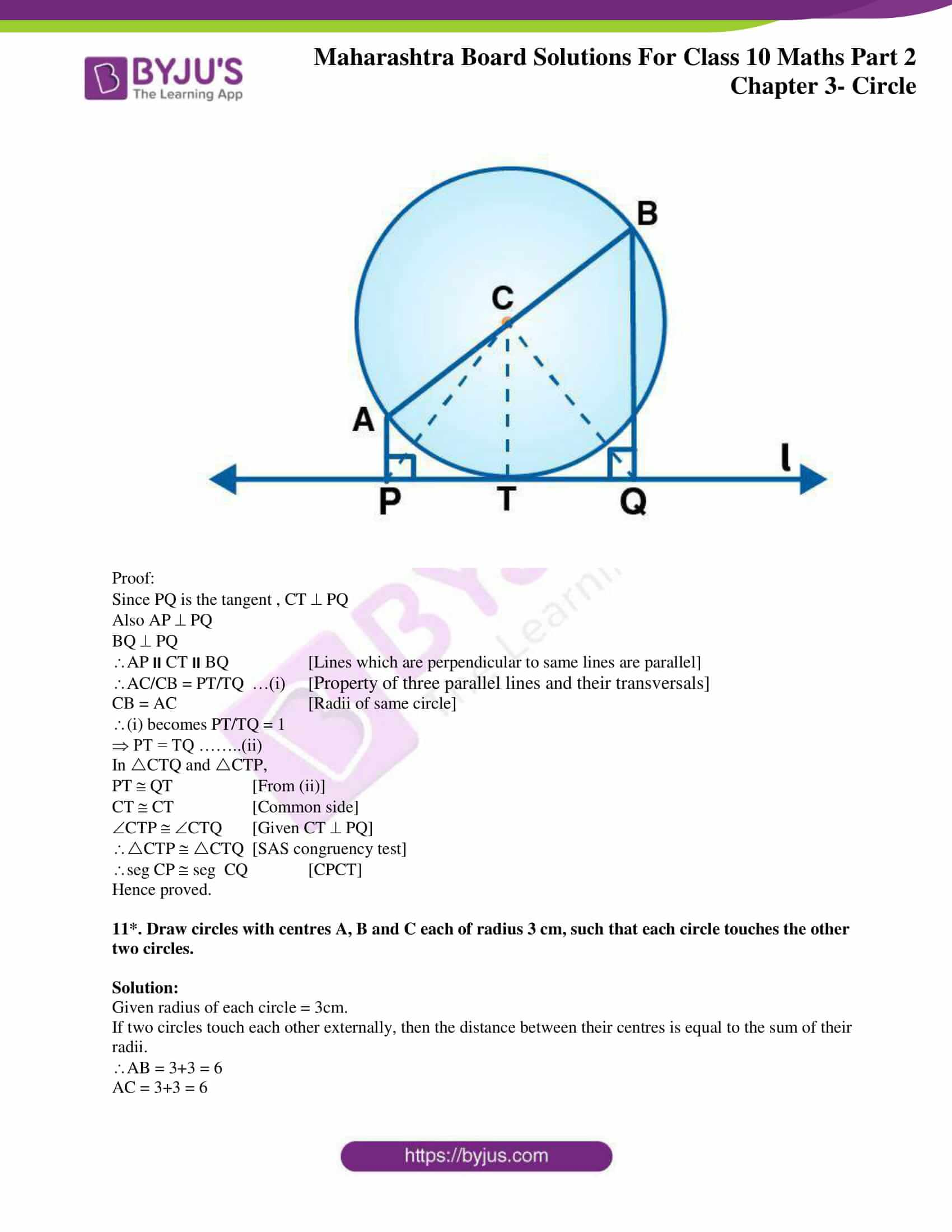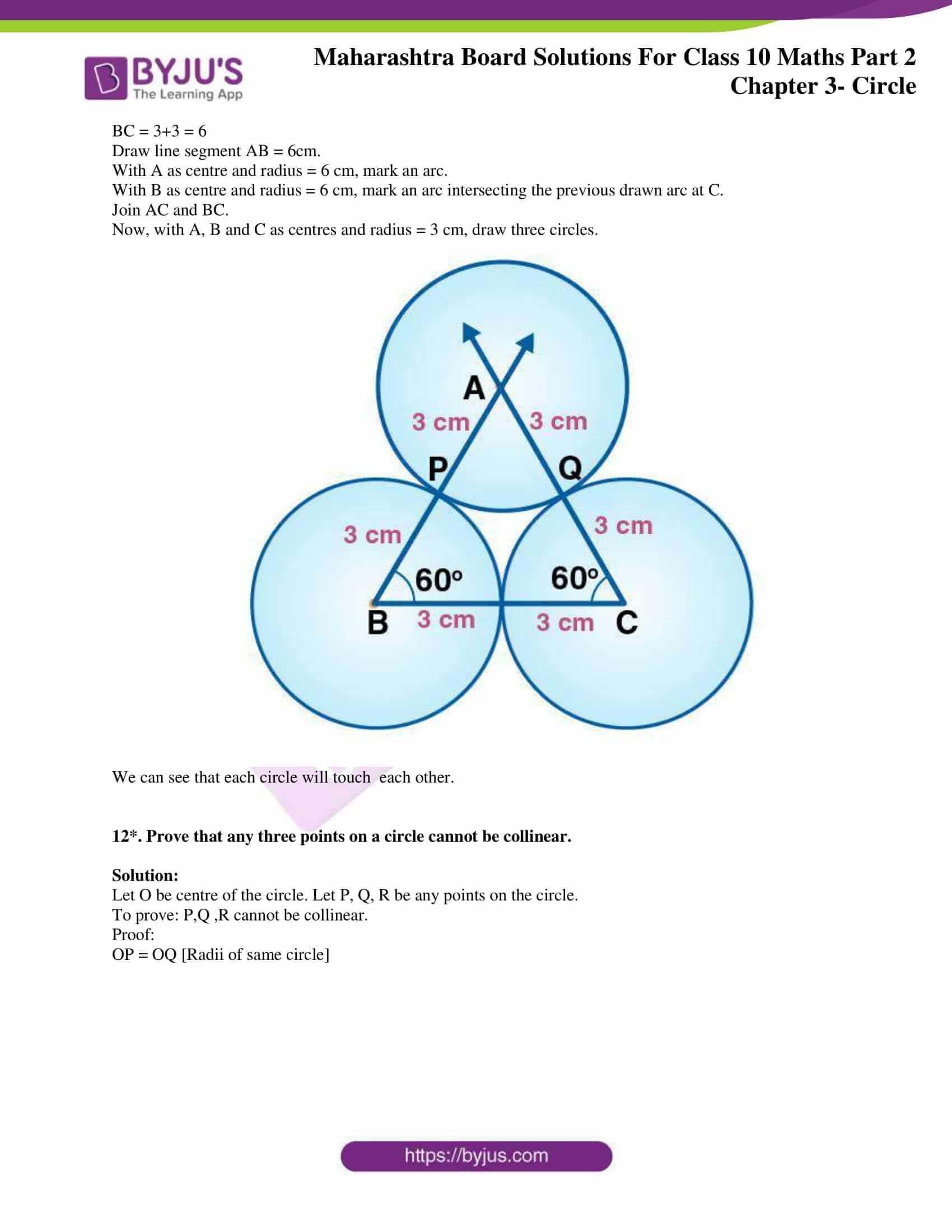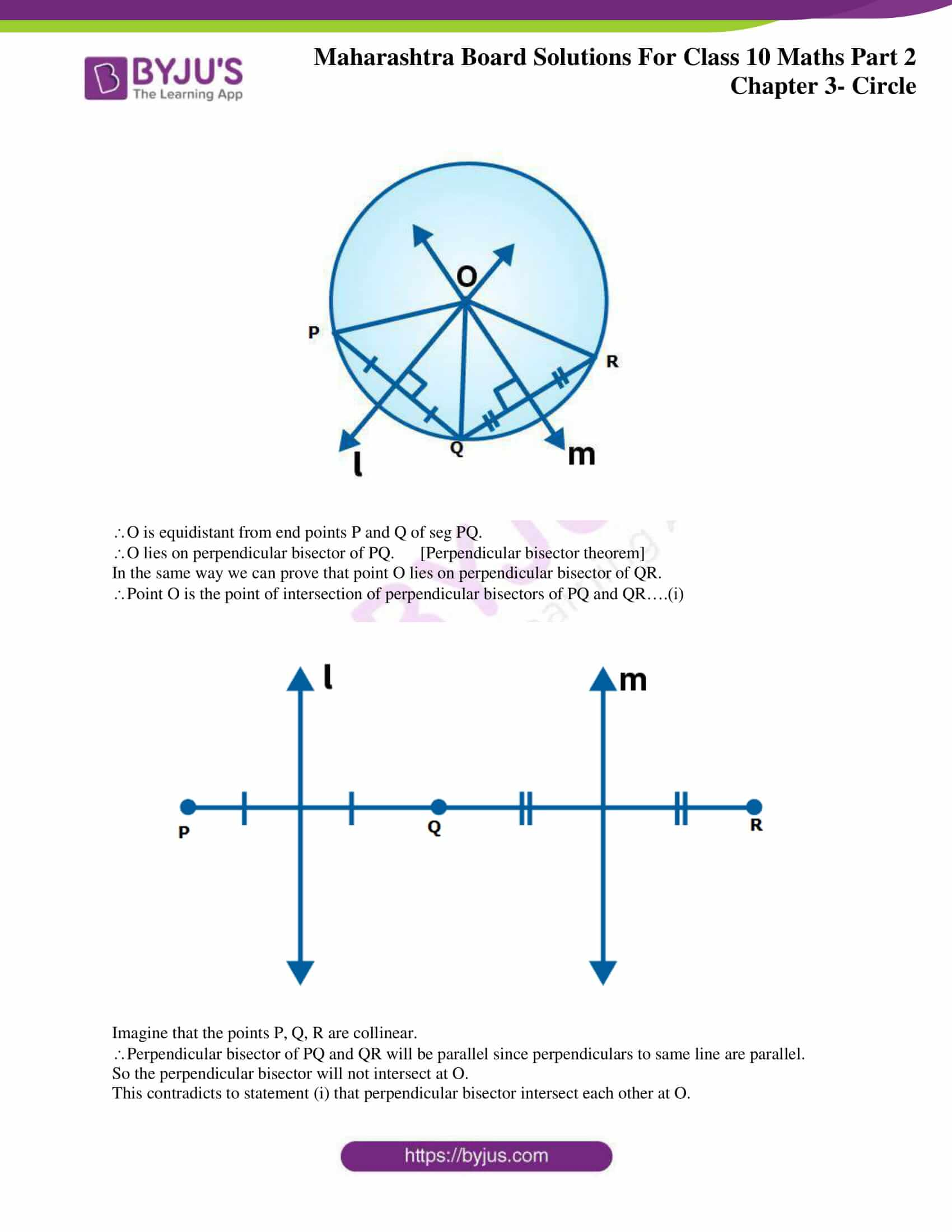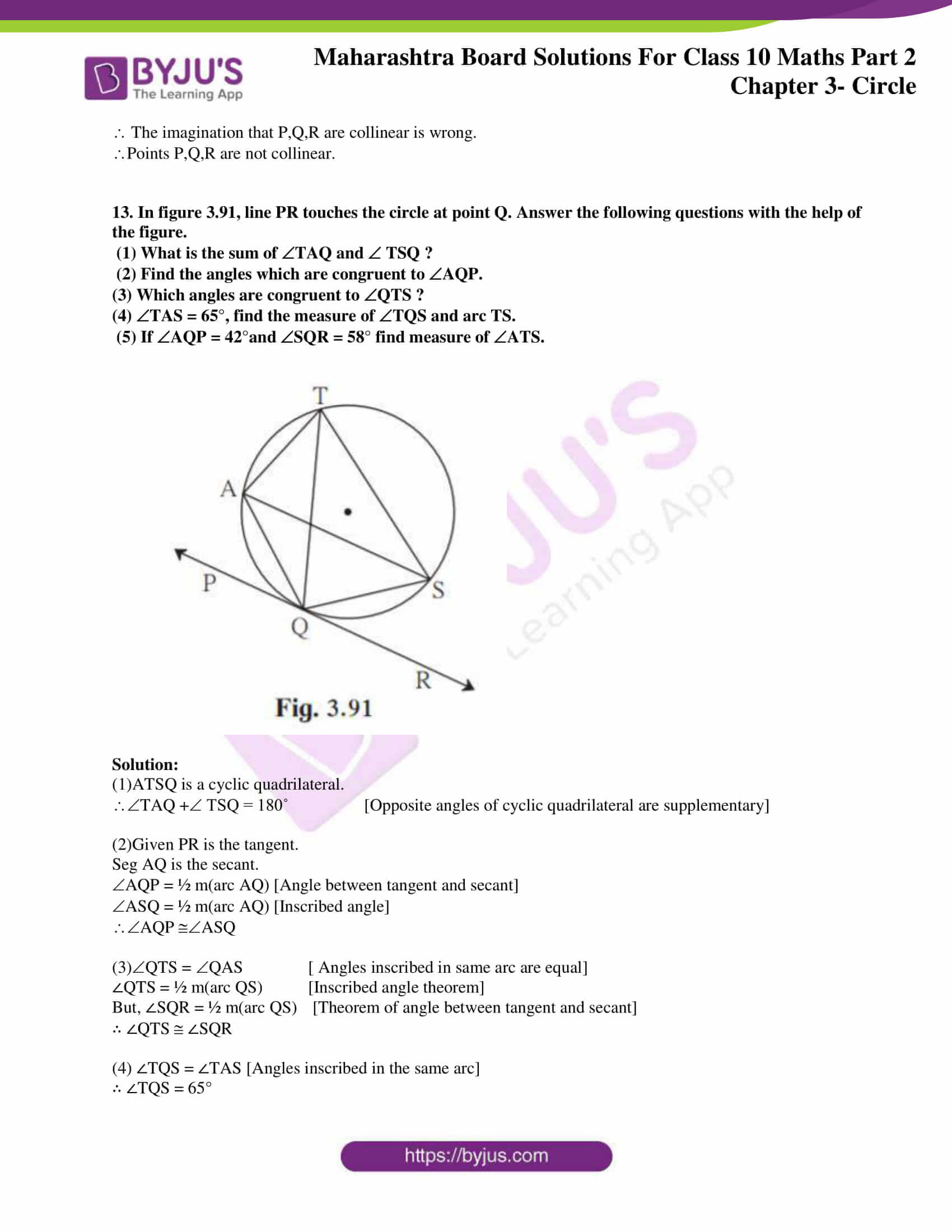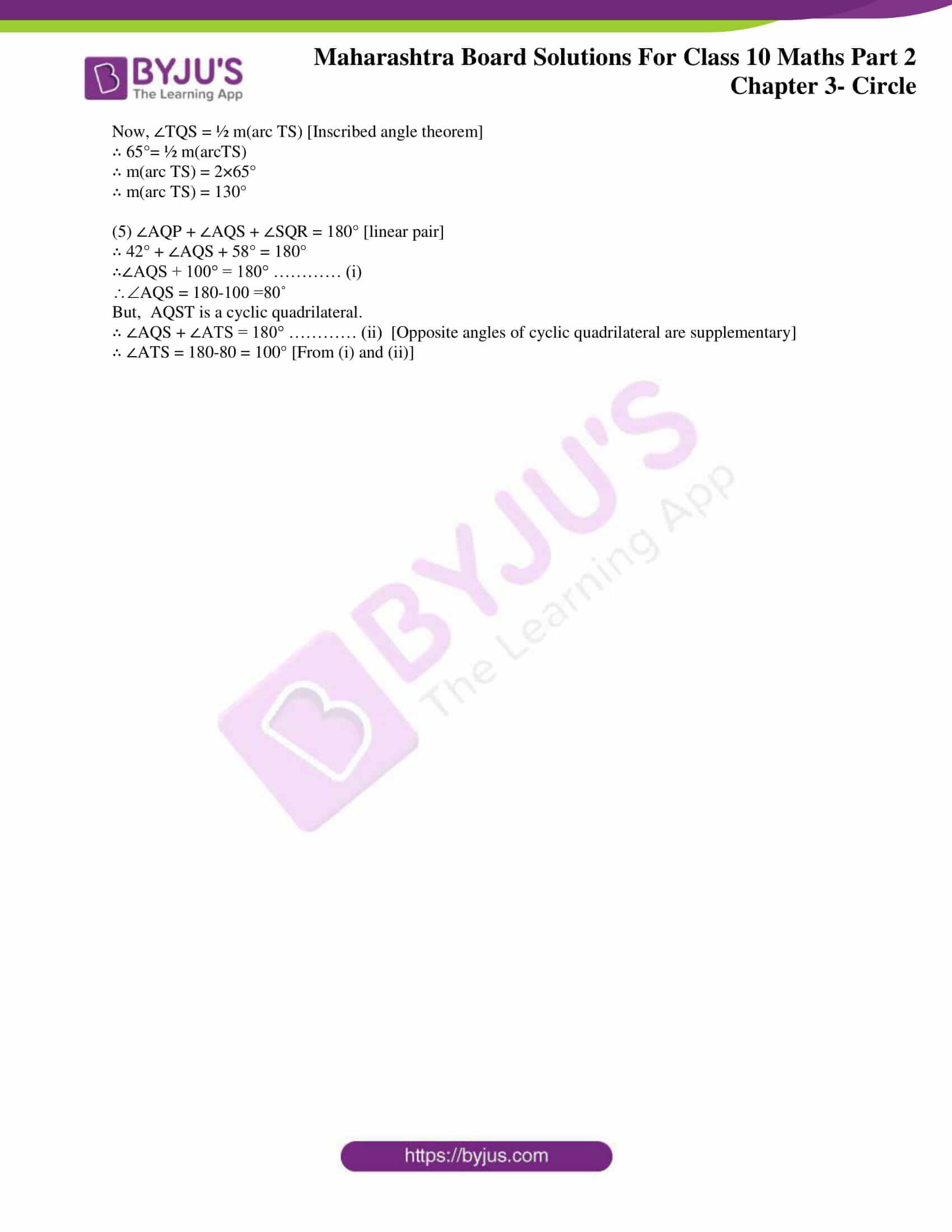### Access answers to Maths MSBSHSE Solutions For SSC Part 2 Chapter 3 – Circle

Practice Set 3.1 Page 55

1. In the adjoining figure the radius of a circle with centre C is 6 cm, line AB is a tangent at A. Answer the following questions.

(1) What is the measure of CAB ? Why ?

(2) What is the distance of point C from line AB? Why ?

(3) d(A,B)= 6 cm, find d(B,C).

(4) What is the measure of ABC ? Why ?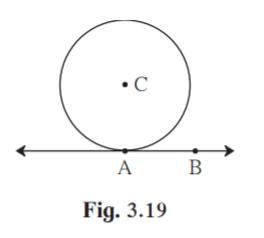Solution:

(1)Given AB is the tangent to the circle with centre C.

CAB = 90˚ [The tangent at any point of a circle is perpendicular to the radius through the point of contact.]

(2) Line AB seg CA. [Tangent theorem]

CA is the radius of the circle.

CA = 6cm

Distance of point C from AB is 6cm.

(3)In CAB , A = 90˚.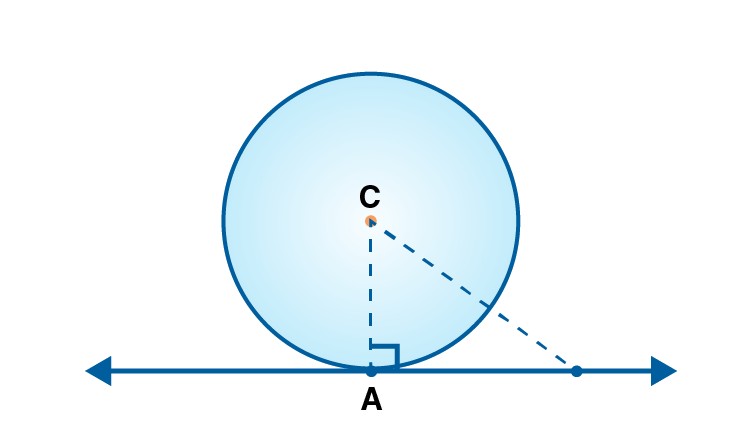AB = 6

BC2 = AC2+AB2 [Pythagoras theorem]

BC2 = 62+62

BC2 = 72

Taking square root on both sides

BC = √72 = 6√2 cm

d(B,C) = 6√2 cm.

(4)InABC , AB = AC = 6

ABC is an isosceles triangle.

Angles opposite two equal sides will be equal in isosceles triangle.

C = B……..(i)

A = 90˚ [Tangent theorem]

A + C +B = 180 [Angle sum property of triangle]

90+ C +B = 180

C +B = 90

C = B = 45˚ [From (i)]

ABC = 45˚

2. In the adjoining figure, O is the centre of the circle. From point R, seg RM and seg RN are tangent segments touching the circle at M and N. If (OR) = 10 cm and radius of the circle = 5 cm, then

(1) What is the length of each tangent segment ?

(2) What is the measure of MRO ?

(3) What is the measure of MRN ?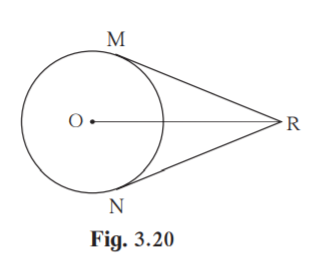Solution:

(1)Given RM and RN are the tangents to the circle.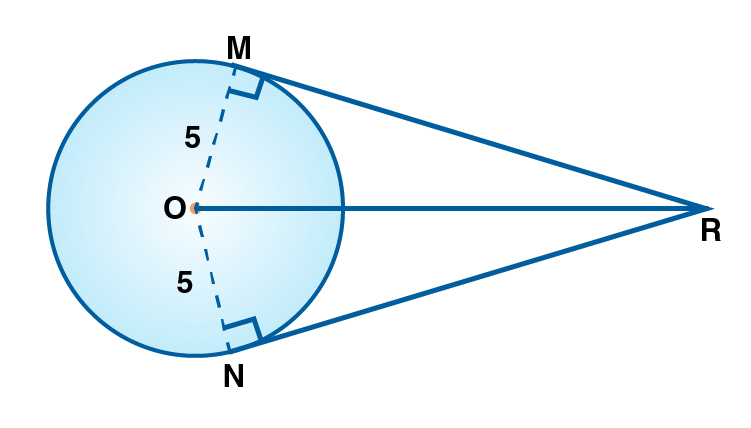OMR = ONR = 90˚ [Tangent theorem]

In OMR , OR = 10 cm , [Given]

OR2 = OM2+MR2 [Pythagoras theorem]

102 = 52+MR2

MR2 = 102-55

MR2 = 75

MR = 5√3

RN = 5√3 [Tangent segments drawn from an external point to a circle are congruent]

(2)In OMR , OMR = 90˚ [Tangent theorem]

OR = 10 cm [Given]

OM = ½ OR

MRO = 30˚ …(i) [Converse of 30˚- 60˚ -90˚ theorem]

NRO = 30˚……..(ii)

(3)MRN = MRO +NRO

MRN = 30+30 = 60˚ [from (i) and (ii)]

Practice set 3.2 Page 58

1. Two circles having radii 3.5 cm and 4.8 cm touch each other internally. Find the distance between their centres.

Solution:

The distance between the centres of the circles touching internally is equal to the difference of their radii.

We have r1 = 3.5 and r2 = 4.8

Distance between their centres = 4.8-3.5 = 1.3 cm.

2. Two circles of radii 5.5 cm and 4.2 cm touch each other externally. Find the distance between their centres.

Solution:

If the circles touch each other externally, distance between their centres is equal to the sum of their radii.

We have r1 = 5.5 and r2 = 4.2

Distance between their centres = 5.5 +4.2 = 9.7 cm

3. If radii of two circles are 4 cm and 2.8 cm. Draw figure of these circles touching each other – (i) externally (ii) internally.

Solution:

(i)Circles touching externally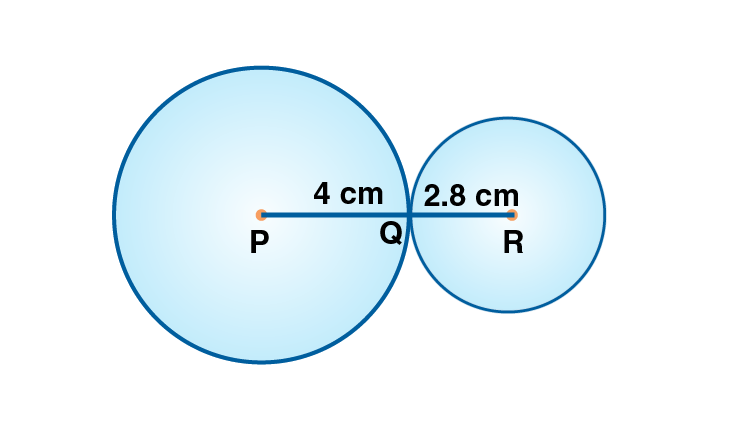If the circles touch each other externally, distance between their centres is equal to the sum of their radii.

Distance between the centres = 4+2.8 = 6.8cm

(ii)Circles touching internally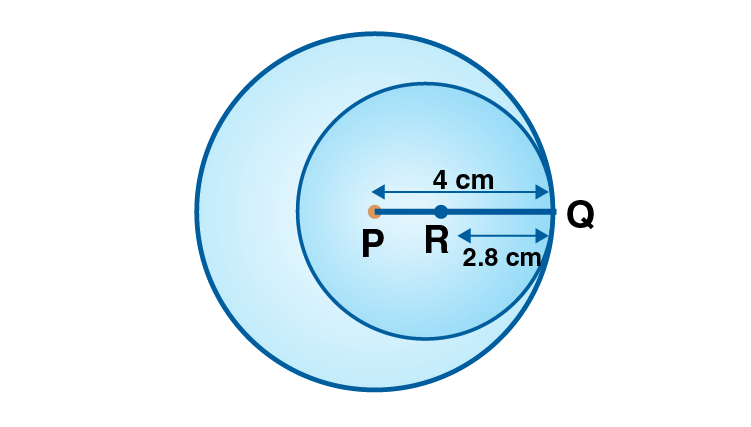The distance between the centres of the circles touching internally is equal to the difference of their radii.

Distance between the centres = 4-2.8 = 1.2cm

Practice set 3.3 Page 63

1. In figure 3.37, points G, D, E, F are concyclic points of a circle with centre C. ECF = 70°, m(arc DGF) = 200° find m(arc DE) and m(arc DEF).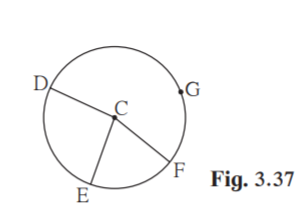Solution:

Given ECF = 70˚

m(arc DGF) = 200˚

m(arc EF) = 70˚ [The measure of a minor arc is the measure of its central angle.]

m(arc DGF)+m(arc EF)+m(arc DE) = 360˚ [Measure of a complete circle is 360°.]

200+70+ m(arc DE) = 360

m(arc DE) = 360-(200+70)

m(arc DE) = 90˚

m(arc DEF) = m(arc DE)+m(arc EF) [Property of sum of measures of arcs ]

m(arc DEF) = 90+70

m(arc DEF) = 160˚

2*. In fig 3.38 QRS is an equilateral triangle. Prove that,

(1) arc RS arc QS arc QR

(2) m(arc QRS) = 240°.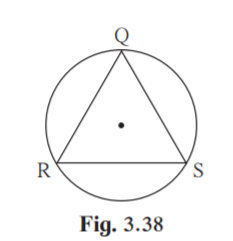Solution:

(1)Given QRS is an equilateral triangle, sides are equal in measure.

QR = RS = QS

arc QR = arc RS = arc QS [Corresponding arcs of congruent chords of a circle are congruent]

arc RS arc QS arc QR….(i)

Hence proved.

(2)m(arc RS)+ m(arc QS)+ m(arc QR) = 360˚ [Measure of a complete circle is 360°]

Also from (i) arc RS arc QS arc QR

Let m(arc RS) = x

x+x+x = 360

3x = 360

x = 120˚

m(arc QRS) = m(arc QR)+m(arc RS) [Property of sum of measures of arcs ]

m(arc QRS) = 120+120 = 240˚

Hence proved.

Practice set 3.4 Page 73

1. In figure 3.56, in a circle with centre O, length of chord AB is equal to the radius of the circle. Find measure of each of the following.

(1) AOB

(2)ACB

(3) arc AB

(4) arc ACB.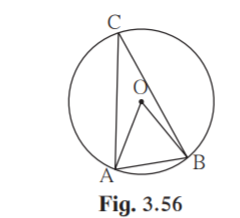Solution:

(1)OA and OB are the radius of circle.

Given AB = radius of circle

AB = OA = OB

OAB is an equilateral triangle.

AOB = 60˚ [Angle of equilateral triangle = 60˚]

(2)ACB = ½ AOB [The measure of an angle subtended by an arc at a point on the circle is half of the measure of the angle subtended by the arc at the centre.]

ACB = ½ ×60 = 30˚

(3)arc AB = AOB [Measure of a minor arc is equal to the measure of its corresponding central angle.]

arc AB = 60˚

(4)m(arc AB) + m(arc ACB) = 360˚ [Measure of a complete circle is 360°]

60+ m(arc ACB) = 360˚

m(arc ACB) = 360-60 = 300˚

Hence arc(ACB ) = 300˚

2. In figure 3.57, PQRS is cyclic. side PQ side RQ. PSR = 110°, Find

(1) measure of PQR

(2) m(arc PQR)

(3) m(arc QR)

(4) measure of PRQSolution:

(1)Given PSR = 110˚

PQR = 180-110 = 70˚ [Opposite angles of a cyclic quadrilateral are supplementary.]

(2)PSR = ½ m(arc PQR) [The measure of an inscribed angle is half the measure of the arc intercepted by it]

m(arc PQR ) = 2×PSR

m(arc PQR ) = 2×110

m(arc PQR ) = 220˚

(3)Given side PQ side RQ

arc PQ arc RQ [Corresponding arcs of congruent chords of a circle are congruent. ]

m(arc PQ) = m(arc RQ)

M(arc PQR ) = m(arc PQ)+m(arc QR) [Property of sum of measures of arcs ]

220 = m(arc PQ)+m(arc QR)

m(arc PQ) = m(arc RQ) = 220/2 = 110˚

(4) m(arc PQ) = 110˚

PRQ = ½ m(arc PQ) [The measure of an inscribed angle is half the measure of the arc intercepted by it]

PRQ = ½ ×110

PRQ = 55˚

3. MRPN is cyclic, R=(5x – 13)°, N=(4x+4)°. Find measures of R and N.

Solution: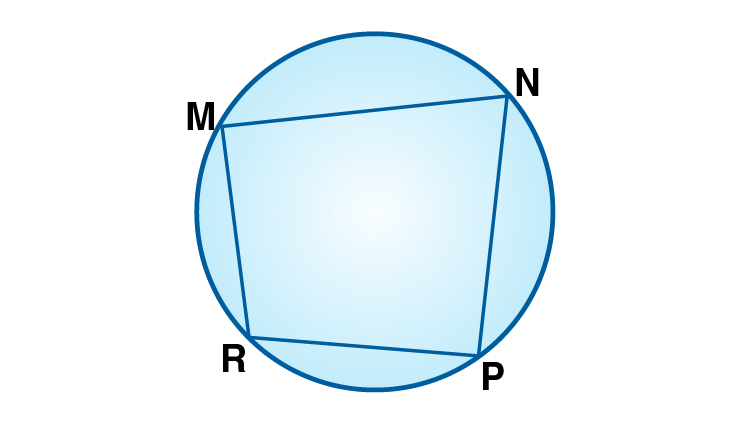Given R=(5x – 13)°, N=(4x+4)°

Opposite angles of a cyclic quadrilateral are supplementary.

R+N = 180˚

5x -13+4x+4 = 180

9x-9 = 180

9x = 189

x = 189/9

x = 21

R = 5x-13

= 5×21-13

= 92˚

N=(4x+4)°

N = 4×21+4

= 84+4

= 88

N = 88˚

Hence the measure of R = 92˚ and N = 88˚.

4. In figure 3.58, seg RS is a diameter of the circle with centre O. Point T lies in the exterior of the circle. Prove that RTS is an acute angle.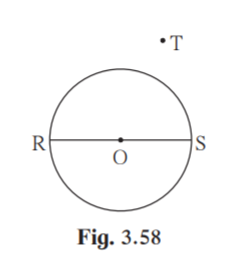Solution:

To prove : RTS is an acute angle.

Construction:

Join RT and ST. Let RT intersect circle at point A. Join AS.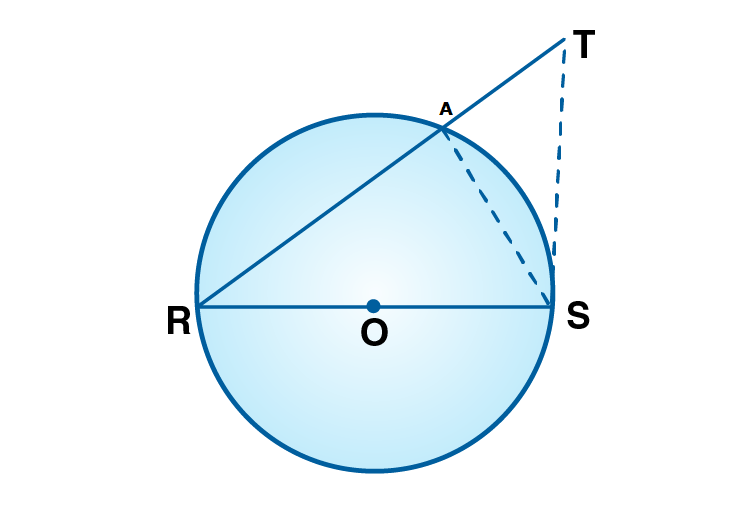Proof:

Given RS is a diameter. O is the centre of the circle.

Since RS is the diameter , RAS = 90˚ [Angle in semi circle is right angle]

In ATS , RAS is an exterior angle and ATS is its remote interior angle.

RAS ATS [Exterior angle of a triangle is greater than remote interior angle]

90˚ATS

ATS 90˚

RTS 90˚ [ATS = RTS ]

RTS is acute.

Hence proved.

Practice set 3.5 Page 82

1. In figure 3.77, ray PQ touches the circle at point Q. PQ = 12, PR = 8, find PS and RS.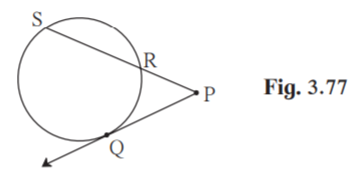Solution:

Given PQ = 12 , PR = 8

PQ is a tangent to the circle.

PQ2 = PR ×PS

122 = 8×PS

PS = 144/8

PS = 18

PS = PR+RS

RS = PS-PR

RS = 18-8 = 10

Hence PS = 18 units and RS = 10 units.

2. In figure 3.78, chord MN and chord RS intersect at point D.

(1) If RD = 15, DS = 4, MD = 8 find DN

(2) If RS = 18, MD = 9, DN = 8 find DS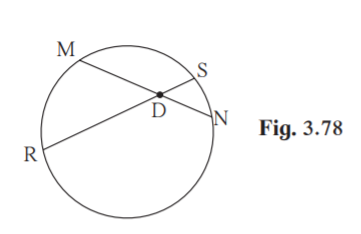Solution:

(1) Given RD = 15, DS = 4, MD = 8

RD×DS = MD×DN [Theorem of chords intersecting inside the circle]

15×4 = 8×DN

DN = 15×4/8 = 60/8

DN = 7.5 units

(2) RS = 18, MD = 9, DN = 8

RD×DS = MD×DN [Theorem of chords intersecting inside the circle]

(RS-DS)×DS = MD×DN [RD +DS = RS]

(18-DS)DS = 9×8

18DS -DS2 = 72

DS2-18DS+72 = 0

Put DS = x

x2-18x+72 = 0

(x-6)(x-12) = 0

x = 6 or x = 12

DS = 6 or DS = 12 units

3. In figure 3.79, O is the centre of the circle and B is a point of contact. seg OE seg AD, AB = 12, AC = 8, find

(2) DC

(3) DE.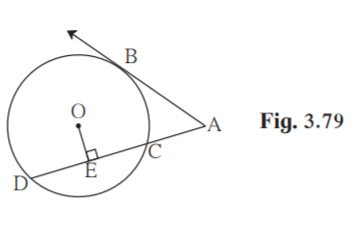Solution:

(1) Given AB = 12 , AC = 8

AB is the tangent . AD is the secant.

AC×AD = AB2 [Tangent secant theorem]

Hence measure of AD is 18 units

18 = 8+DC

DC = 18-8

DC = 10

Hence measure of DC is 10 units.

OECD [A-C-D]

DE = ½ CD [Perpendicular from centre of circle to chord bisects the chord]

DE = ½ ×10

DE = 5

Hence measure of DE is 5 units.

Problem set 3 Page 83

1.Four alternative answers for each of the following questions are given. Choose the correct alternative.

(1) Two circles of radii 5.5 cm and 3.3 cm respectively touch each other. What is the distance between their centers ?

(A) 4.4 cm (B) 8.8 cm (C) 2.2 cm (D) 8.8 or 2.2 cm

Solution:

If the circles touch each other externally, distance between their centres is equal to the sum of their radii.

Distance between the centres = 5.5+3.3 = 8.8cm

The distance between the centres of the circles touching internally is equal to the difference of their radii.

Distance between the centres = 5.5-3.3 = 2.2cm

Hence Option D is the answer.

(2) Two circles intersect each other such that each circle passes through the centre of the other. If the distance between their centres is 12, what is the radius of each circle ?

(A) 6 cm (B) 12 cm (C) 24 cm (D) can’t say

Solution: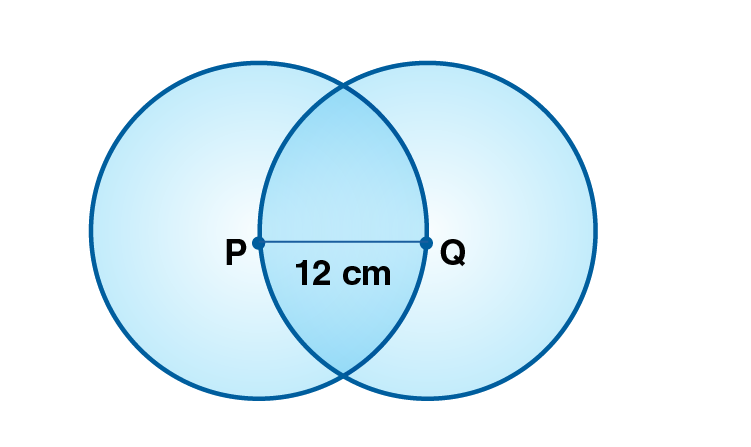Let A and B be centres of two circles.

Then radius of circle with centre A = radius of circle with centre B = Distance between their centres = 12 cm

Hence Option B is the answer.

(3) A circle touches all sides of a parallelogram. So the parallelogram must be a, ………………. .

(A) rectangle (B) rhombus (C) square (D) trapezium

Solution:

It will be a rhombus because rhombus is a parallelogram with all sides equal.

Hence Option B is the answer.

(4) Length of a tangent segment drawn from a point which is at a distance 12.5 cm from the centre of a circle is 12 cm, find the diameter of the circle.

(A) 25 cm (B) 24 cm (C) 7 cm (D) 14 cm

Solution: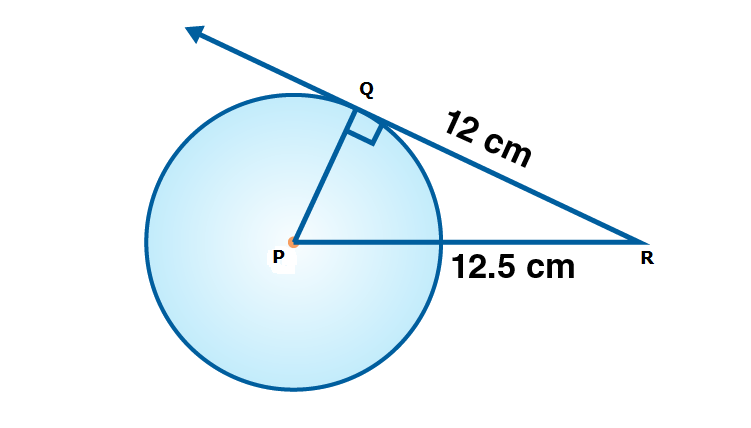In PQR , Q = 90˚ [Tangent theorem]

PR2 = PQ2+QR2 [Pythagoras theorem]

12.52 = PQ2+122

PQ2 = 12.52-122

PQ2 = 156.25-144 = 12.25

PQ = √12.25 = 3.5

Diameter = 2×3.5 = 7cm

Hence Option C is the answer.

(5) If two circles are touching externally, how many common tangents of them can be drawn?

(A) One (B) Two (C) Three (D) Four

Solution: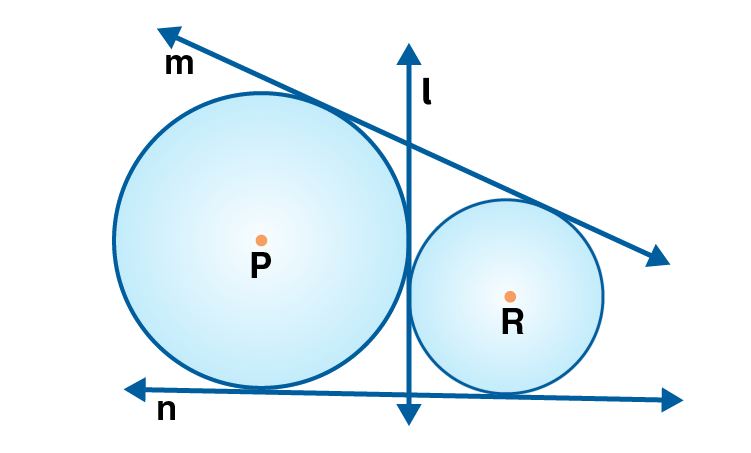If two circles touch each other externally, then three common tangents can be drawn to the circles.

Hence Option C is the answer.

2. Line l touches a circle with centre O at point P. If radius of the circle is 9 cm, answer the following.

(1) What is d(O, P) = ? Why ?

(2) If d(O, Q) = 8 cm, where does the point Q lie ?

(3) If d(O,R) = 15 cm, How many locations of point R are line on line l ? At what distance will each of them be from point P ?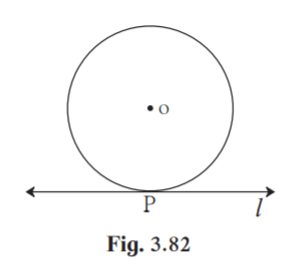Solution:

(1) Given radius = 9cm. Line l is the tangent to the circle.

d(O,P) = 9cm

OP is the radius of circle.

(2) d(O,Q) = 8cm.

d(O,Q) is less than radius. So Q will lie inside circle.

(3) Point R can be on two locations on line l.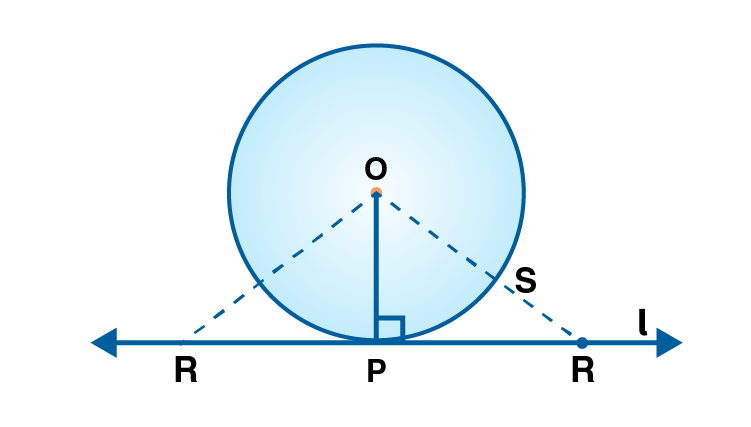d(O,R ) = 15

InOPR, OPR = 90˚ [Tangent theorem]

OP2+PR2 = OR2 [Pythagoras theorem]

92+PR2 = 152

PR2 = 152-92

PR2 = 225-81 = 144

PR = √144 = 12

The point R will be at 12 cm distance from P.

3. In figure 3.83, M is the centre of the circle and seg KL is a tangent segment. If MK = 12, KL = 6√3 then find –

(1) Radius of the circle. (2) Measures of K and M.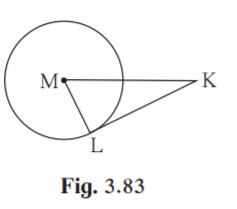Solution:

(1) Given KL is the tangent. ML is the radius.

MLK = 90˚ [Tangent theorem]

MK = 12, KL = 6√3

In MLK, ML2+KL2 = MK2 [Pythagoras theorem]

ML2 = 122-(6√3)2 = 144-108 = 36

ML = 6

Hence radius of the circle is 6cm.

(2) ML = ½ MK

K = 30˚ [Converse of 30˚-60˚-90˚ theorem]

M = 180-(90+30) = 60˚ [Angle sum property]

Hence K = 30˚ and M = 60˚

4. In figure 3.84, O is the centre of the circle. Seg AB, seg AC are tangent segments. Radius of the circle

is r and l(AB) = r, Prove that, ABOC is a square.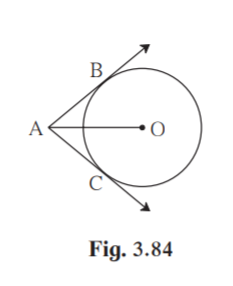Solution:

Construction: Draw OC and OB.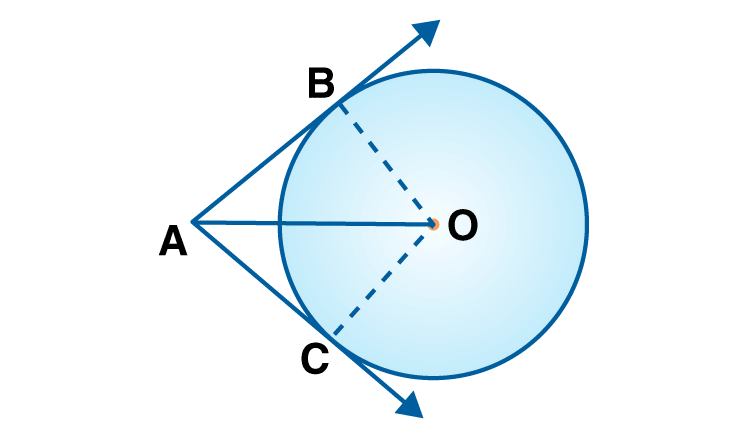Proof:

Given AB and AC are the tangents and r is the radius of the circle.

AB = AC …….(i) [Two tangents from a common point are congruent]

OB = OC = r …(ii) [radii of same circle]

Given AB = r

AB = AC =OB = OC [From (i) and (ii)]

OBA = OCA = 90˚ [Tangent theorem]

ABOC is a square.

5. In figure 3.85, ABCD is a parallelogram. It circumscribes the circle with centre T. Point E, F, G, H

are touching points. If AE = 4.5, EB = 5.5, find AD.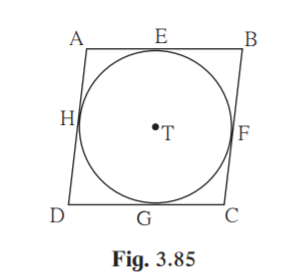Solution: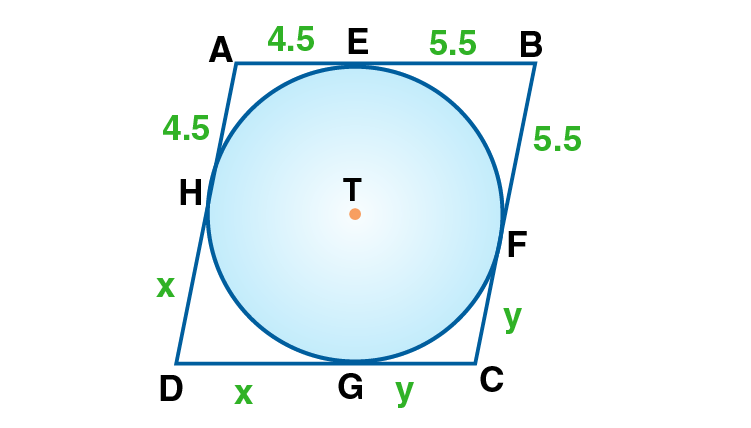Given ABCD is a parallelogram.

AB = DC ……(i) [Opposite sides of parallelogram are equal]

AE = AH………(iii) [Two tangents from a common point are congruent]

BE = BF……….(iv)

CG = CF………..(v)

DG = DH………..(vi)

AE+BE+CG+DG = AH+BF+CF+DH

From (i), (ii) and (vii)

AD = AB = AE +EB = 4.5+5.5 = 10

Hence measure of AD is 10 units.

6. In figure 3.86, circle with centre M touches the circle with centre N at point T. Radius RM touches

the smaller circle at S. Radii of circles are 9 cm and 2.5 cm. Find the answers to the following questions

hence find the ratio MS:SR.

(1) Find the length of segment MT

(2) Find the length of seg MN

(3) Find the measure of NSM.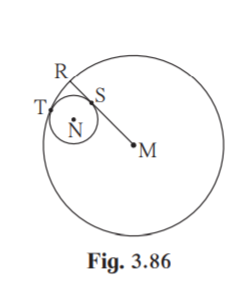Solution:

(1) Given radius of bigger circle is 9cm.

Length of segment MT = 9cm.

(2) MT = MN+NT [M-N-T]

9 = MN+2.5 [Given radius of smaller circle is 2.5]

MN = 9-2.5

MN = 6.5cm.

(3) RM touches smaller circle at S.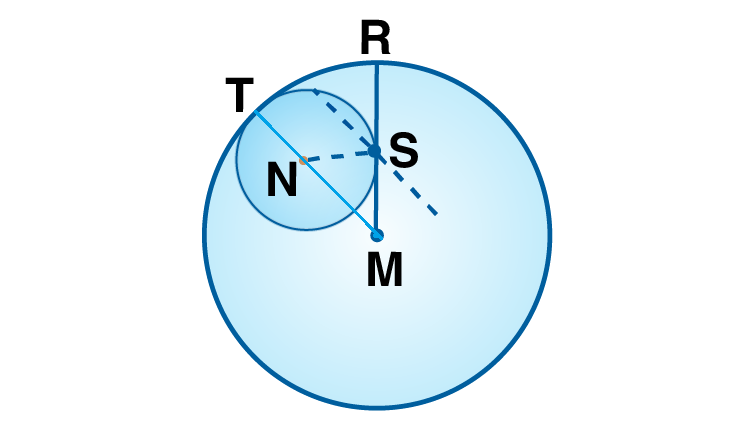MR is the tangent to the smaller circle. NS is the radius of smaller circle.

NSM = 90˚ [Tangent theorem]

In NSM , NS2+MS2 = MN2

2.52+MS2 = 6.52

MS2 = 6.52-2.52

MS2 = 42.25-6.25

MS2 = 36

MS = 6

MR = SR+SM [R-S-M]

9 = SR+6

SR = 9-6 = 3

MS:SR = 6:3 = 2:1

7. In the adjoining figure circles with centres X and Y touch each other at point Z. A secant passing

through Z intersects the circles at points A and B respectively. Prove that, radius XA || radius YB. Fill

in the blanks and complete the proof.

Construction: Draw segments XZ and ………. .

Proof: By theorem of touching circles, points X, Z, Y are ………. .

XZA ………. opposite angles

Let XZA = BZY = a ….. (I)

Now, seg XA seg XZ …….. (……….)

XAZ = ………. = a …….. (isosceles triangle theorem) (II)

similarly, seg YB ………. …….. (……….)

BZY = ………. = a …….. (……….) (III)

from (I), (II), (III),

XAZ = ……….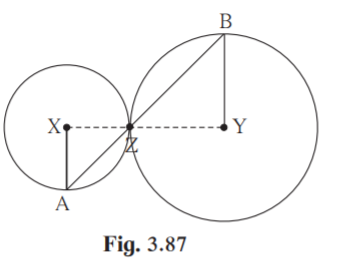Solution:

Construction: Draw segments XZ and YZ

Proof: By theorem of touching circles, points X, Z, Y are collinear.

XZA BZY vertically opposite angles

Let XZA = BZY = a ….. (I)

Now, seg XA seg XZ …….. (Radii of same circle)

XAZ = XZA. = a …….. (isosceles triangle theorem) (II)

similarly, seg YB seg YZ (Radii of same circle)

BZY = ZBY = a …….. (isosceles triangle theorem) (III)

from (I), (II), (III),

XAZ = ZBY

8. In figure 3.88, circles with centres X and Y touch internally at point Z . Seg BZ is a chord of bigger

circle and it itersects smaller circle at point A. Prove that, seg AX || seg BY.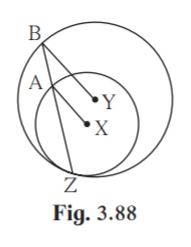Solution:

Given X and Y are the centres of the circle.

Proof:

Join YZ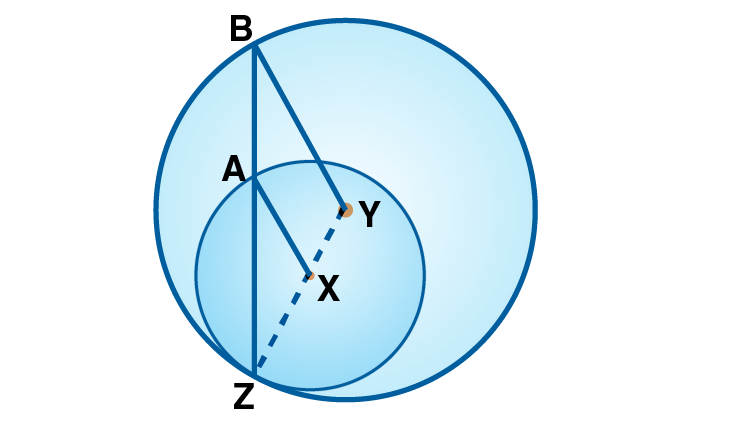In AXZ ,

AX = XZ [Radii of same circle]

XAZ = XZA….(i) [Isoceles triangle theorem]

In BYZ,

YB = YZ [Radii of same circle]

YBZ = YZB [Isoceles triangle theorem]

XZA = YBZ ..(ii) [Y-X-Z, B-A-Z]

From (i) and (ii)

XAZ = YBZ

If a pair of corresponding angles formed by a transversal on two lines is congruent, then the two lines are

parallel.

seg AX seg BY [corresponding angles test]

Hence proved.

9. In figure 3.89, line l touches the circle with centre O at point P. Q is the mid point of radius OP. RS is

a chord through Q such that chords RS || line l. If RS = 12 find the radius of the circle.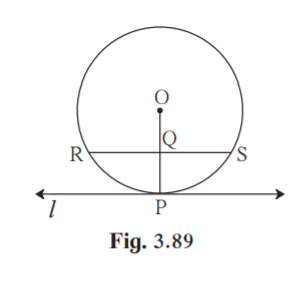Solution: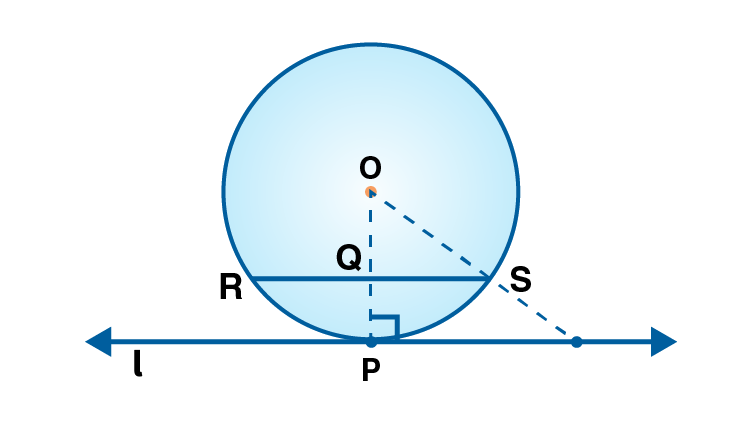Given line l is a tangent.

Let the radius of circle be r.

OP line l. [Tangent theorem]

Given chord RSline l.

OP chord RS

Since the perpendicular from centre of the circle to the chord bisects the chord,

QS = ½ RS

QS = ½ ×12 = 6

OQ = r/2 [Given Q is the midpoint of OP]

In OQS

OS2 = OQ2+QS2 [Pythagoras theorem]

r2 = (r/2)2+62

r2-r2/4 = 36

(3/4)r2 = 36

r2 = 36×4/3

r2= 48

r = 4√3

Hence radius of circle is 4√3cm.

10*.In figure 3.90, seg AB is a diameter of a circle with centre C. Line PQ is a tangent, which touches

the circle at point T. seg AP line PQ and seg BQ line PQ. Prove that, seg CP seg CQ.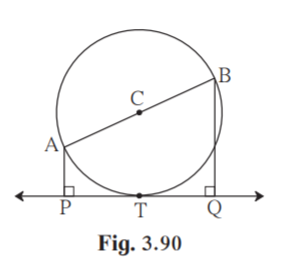Solution:

Given AB is the diameter of the circle with centre C.

PQ is the tangent.

AP PQ

BQPQ

To prove seg CP seg CQ

Construction: Join CP, CT and CQ.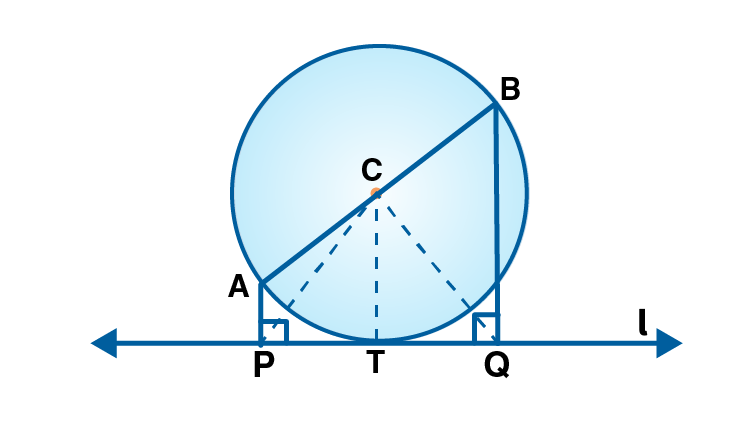Proof:

Since PQ is the tangent , CT PQ

Also AP PQ

BQ PQ

APCTBQ [Lines which are perpendicular to same lines are parallel]

AC/CB = PT/TQ …(i) [Property of three parallel lines and their transversals]

CB = AC [Radii of same circle]

(i) becomes PT/TQ = 1

PT = TQ ……..(ii)

In CTQ and CTP,

PT QT [From (ii)]

CT CT [Common side]

CTP CTQ [Given CT PQ]

CTP CTQ [SAS congruency test]

seg CP seg CQ [CPCT]

Hence proved.

11*. Draw circles with centres A, B and C each of radius 3 cm, such that each circle touches the other

two circles.

Solution:

Given radius of each circle = 3cm.

If two circles touch each other externally, then the distance between their centres is equal to the sum of their

AB = 3+3 = 6

AC = 3+3 = 6

BC = 3+3 = 6

Draw line segment AB = 6cm.

With A as centre and radius = 6 cm, mark an arc.

With B as centre and radius = 6 cm, mark an arc intersecting the previous drawn arc at C.

Join AC and BC.

Now, with A, B and C as centres and radius = 3 cm, draw three circles.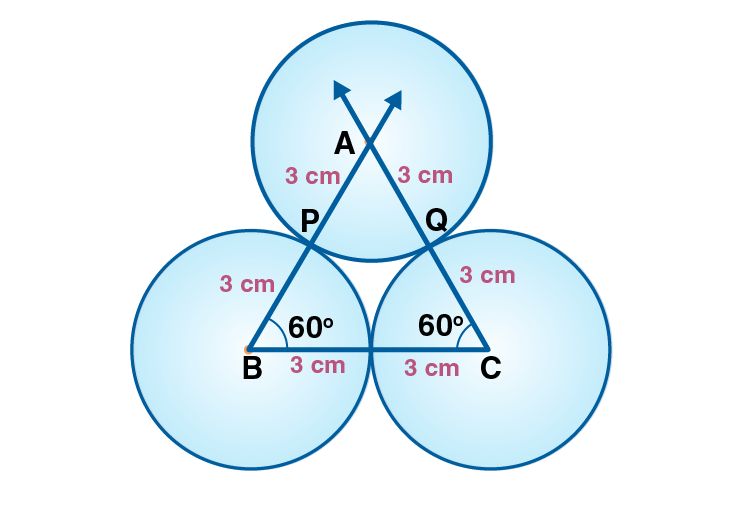We can see that each circle will touch each other.

12*. Prove that any three points on a circle cannot be collinear.

Solution:

Let O be centre of the circle. Let P, Q, R be any points on the circle.

To prove: P,Q ,R cannot be collinear.

Proof:

OP = OQ [Radii of same circle]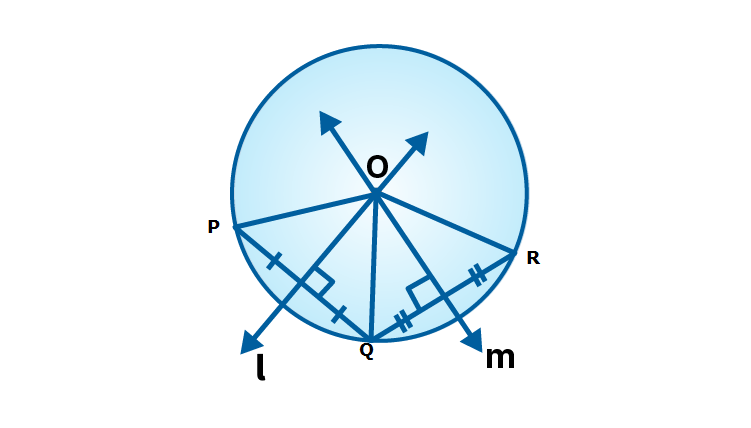O is equidistant from end points P and Q of seg PQ.

O lies on perpendicular bisector of PQ. [Perpendicular bisector theorem]

In the same way we can prove that point O lies on perpendicular bisector of QR.

Point O is the point of intersection of perpendicular bisectors of PQ and QR….(i)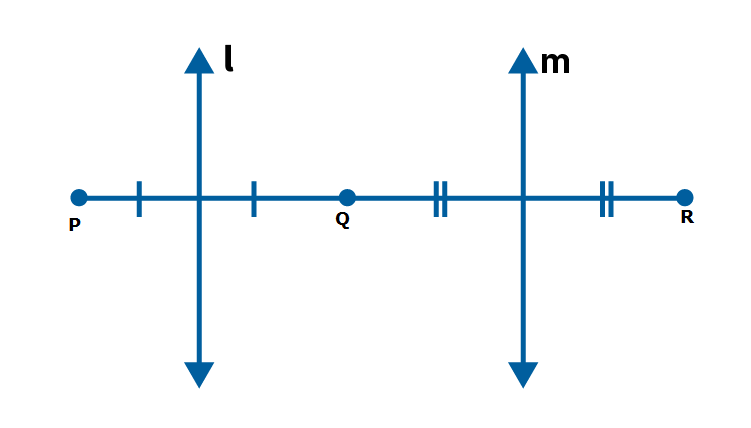Imagine that the points P, Q, R are collinear.

Perpendicular bisector of PQ and QR will be parallel since perpendiculars to same line are parallel.

So the perpendicular bisector will not intersect at O.

This contradicts to statement (i) that perpendicular bisector intersect each other at O.

The imagination that P,Q,R are collinear is wrong.

Points P,Q,R are not collinear.

13. In figure 3.91, line PR touches the circle at point Q. Answer the following questions with the help of the figure.

(1) What is the sum of TAQ and TSQ ?

(2) Find the angles which are congruent to AQP.

(3) Which angles are congruent to QTS ?

(4) TAS = 65°, find the measure of TQS and arc TS.

(5) If AQP = 42°and SQR = 58° find measure of ATS.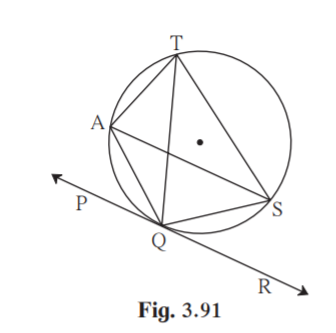Solution:

TAQ + TSQ = 180˚ [Opposite angles of cyclic quadrilateral are supplementary]

(2)Given PR is the tangent.

Seg AQ is the secant.

AQP = ½ m(arc AQ) [Angle between tangent and secant]

ASQ = ½ m(arc AQ) [Inscribed angle]

AQP ASQ

(3)QTS = QAS [ Angles inscribed in same arc are equal]

∠QTS = ½ m(arc QS) [Inscribed angle theorem]

But, ∠SQR = ½ m(arc QS) [Theorem of angle between tangent and secant]

∴ ∠QTS ∠SQR

(4) ∠TQS = ∠TAS [Angles inscribed in the same arc]

∴ ∠TQS = 65°

Now, ∠TQS = ½ m(arc TS) [Inscribed angle theorem]

∴ 65°= ½ m(arcTS)

∴ m(arc TS) = 2×65°

∴ m(arc TS) = 130°

(5) ∠AQP + ∠AQS + ∠SQR = 180° [linear pair]

∴ 42° + ∠AQS + 58° = 180°

∴∠AQS + 100° = 180° ………… (i)

AQS = 180-100 =80˚

But, AQST is a cyclic quadrilateral.

∴ ∠AQS + ∠ATS = 180° ………… (ii)  [Opposite angles of cyclic quadrilateral are supplementary]

∴ ∠ATS = 180-80 = 100° [From (i) and (ii)]

In Class 10 Maths chapter Circle, we learn secant tangent angle theorem and concepts on inscribed angle and intercepted arc. Also, ideas about circles passing through one, two, three points and circles touching each other are found in this chapter. Visit BYJU’S to get more information on concepts and a greater level of understanding Circles, theorems and problems.

## Frequently Asked Questions on Maharashtra State Board Solutions for Class 10 Maths Part 2 Chapter 3 Circle

### Are Maharashtra Board Class 10 Maths Part 2 Chapter 3 Solutions useful?

Yes, these solutions are very useful. Students are highly recommended to practise these solutions after revising the subjects, as they set the basis for the questions that could get asked often in the board exams. Students are encouraged to practise these questions at first. Then they can refer back to the solutions to analyse their performance. This will also help them to rectify the mistakes, so that they can avoid making any during the board exams.

### What are some of the concepts covered in Maharashtra Board Class 10 Maths Part 2 Chapter 3 solutions?

Maharashtra Board Class 10 Maths Part 2 Chapter 3 solutions deals with circles touching each other, secant and tangent of circle, inscribed angle, intercepted arc and arc of a circle. We also come across cyclic quadrilateral and Theorem of intersecting chords in this chapter.

### Are these Maharashtra Board Class 10 Maths Part 2 Chapter 3 solutions downloadable?

Yes, Maharashtra Board Class 10 Maths Part 2 Chapter 3 solutions can be downloaded from the link given. We also provide the Scrollable PDF on the page and the questions and answers online on the webpage.